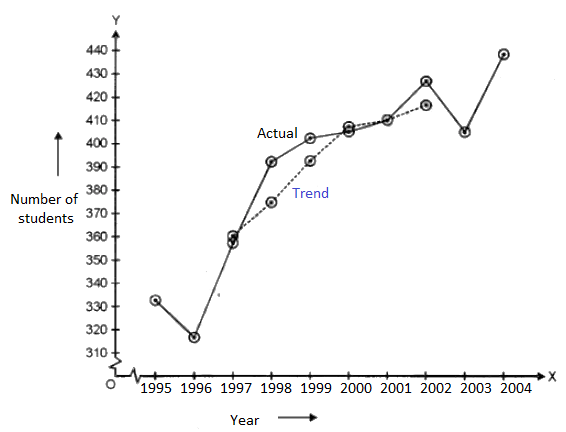ISC Class 11 Maths Sample Question Paper – Mock 2 with Solutions can be accessed in a free downloadable pdf at BYJU’S. These can be referred to online along with the pdf at any time. Students are advised to practise mock sample papers of ISC Class 11 Maths to score maximum marks in the examination. They can also get the solutions of these sample papers to refer as well as to verify once they solve all the questions in ISC Class 11 maths sample papers.

Students can crack Class 11 ISC Maths by memorizing all the formulas of the concepts and important methods of solving problems. Practising more sample papers will help the students in better understanding of the pattern of examination.

## ISC Class 11 Maths Mock Sample Question Paper 2 with Solutions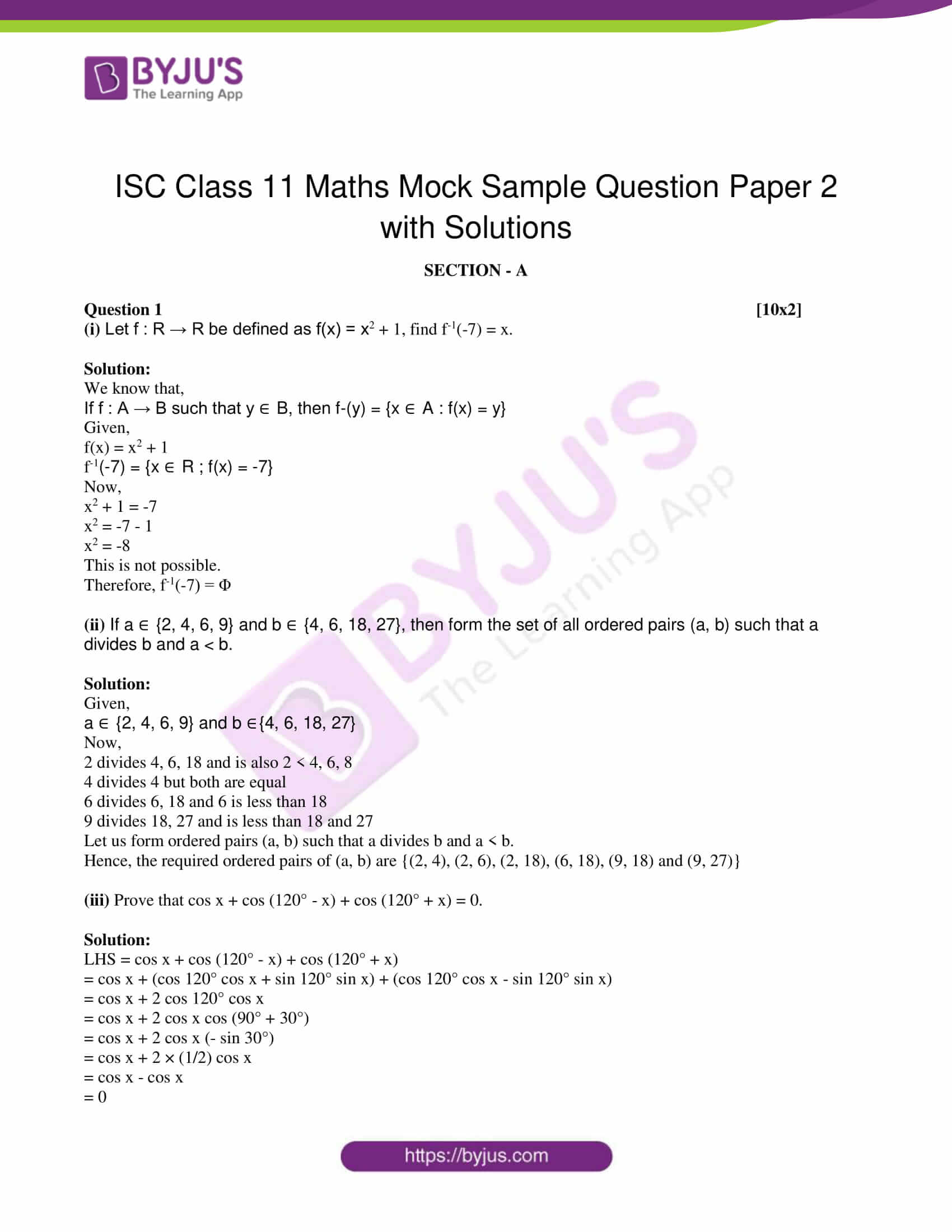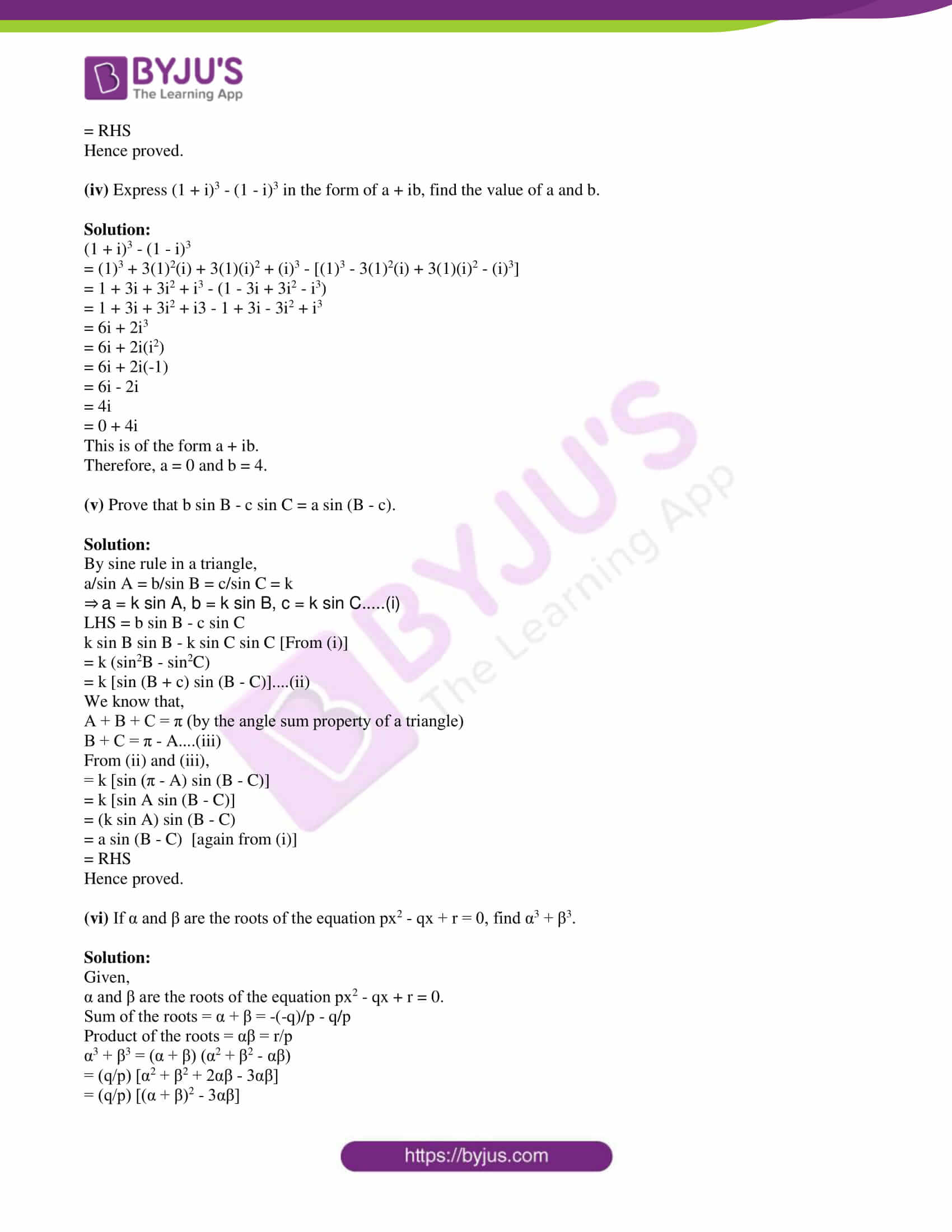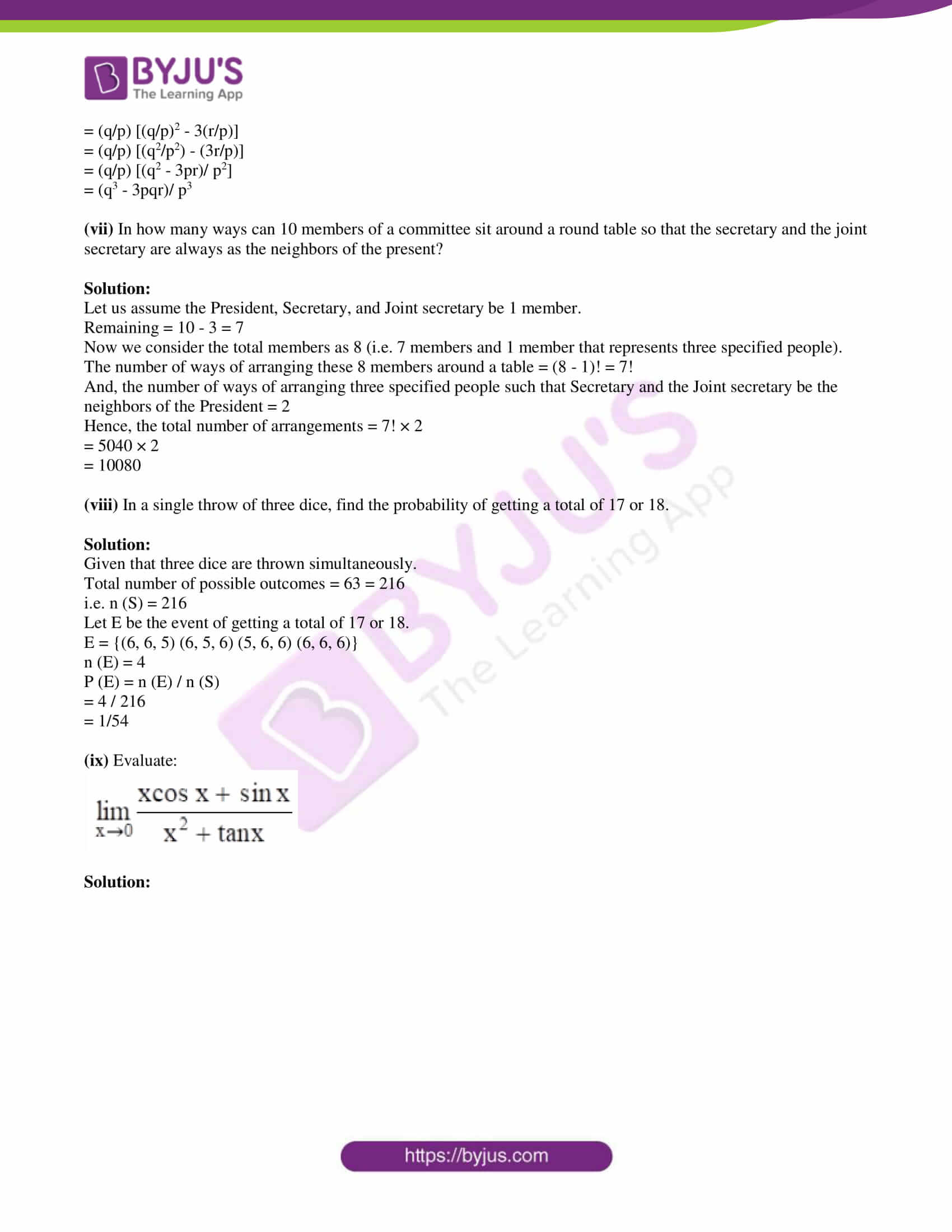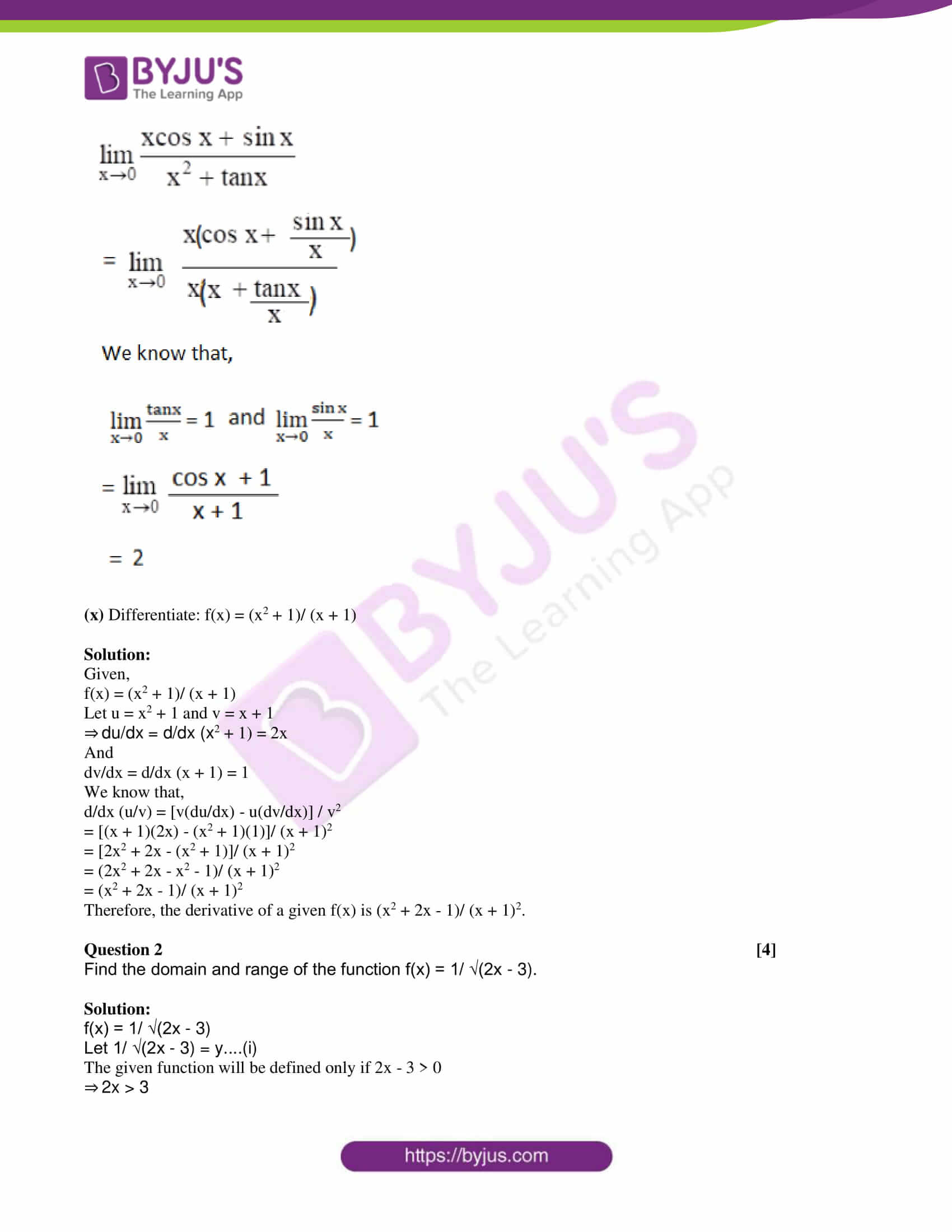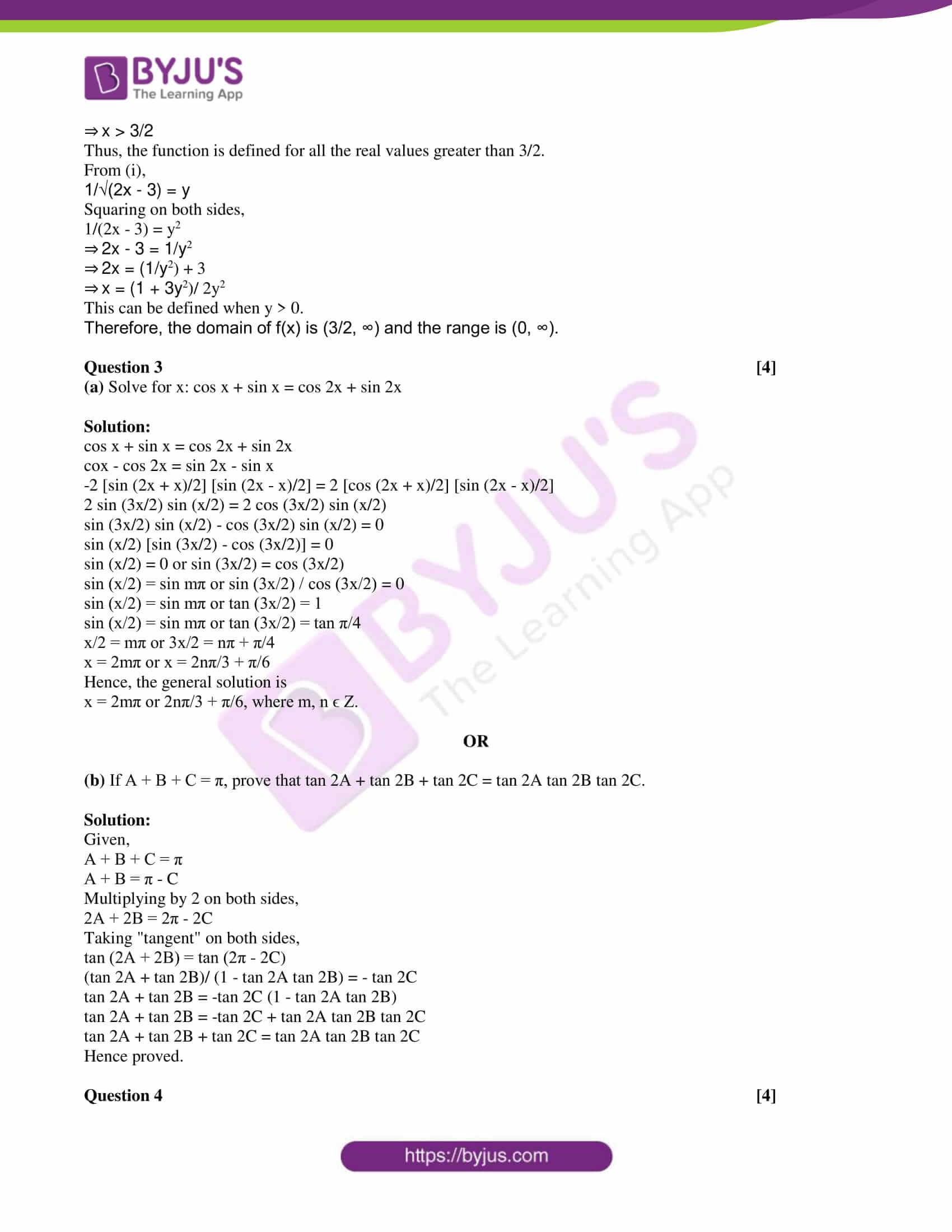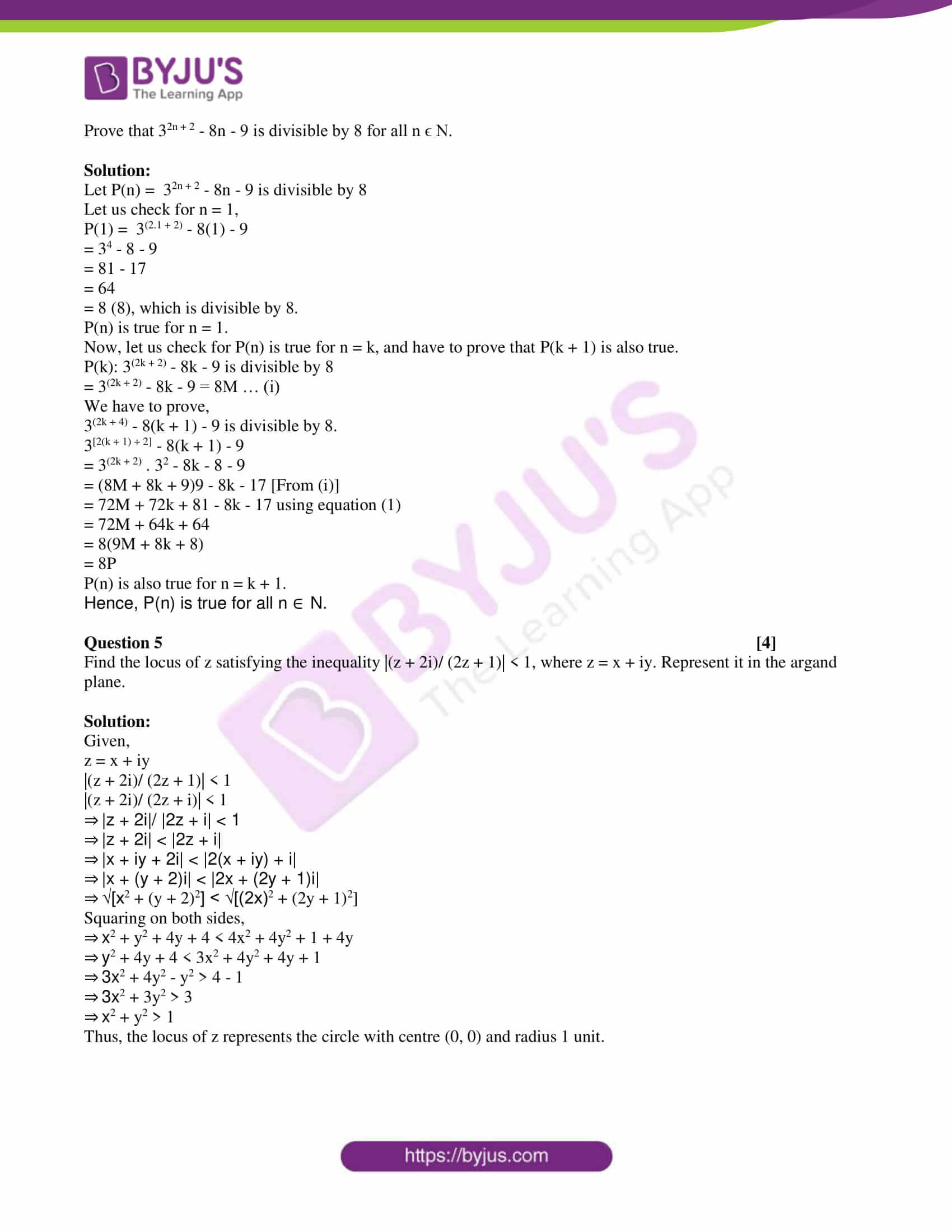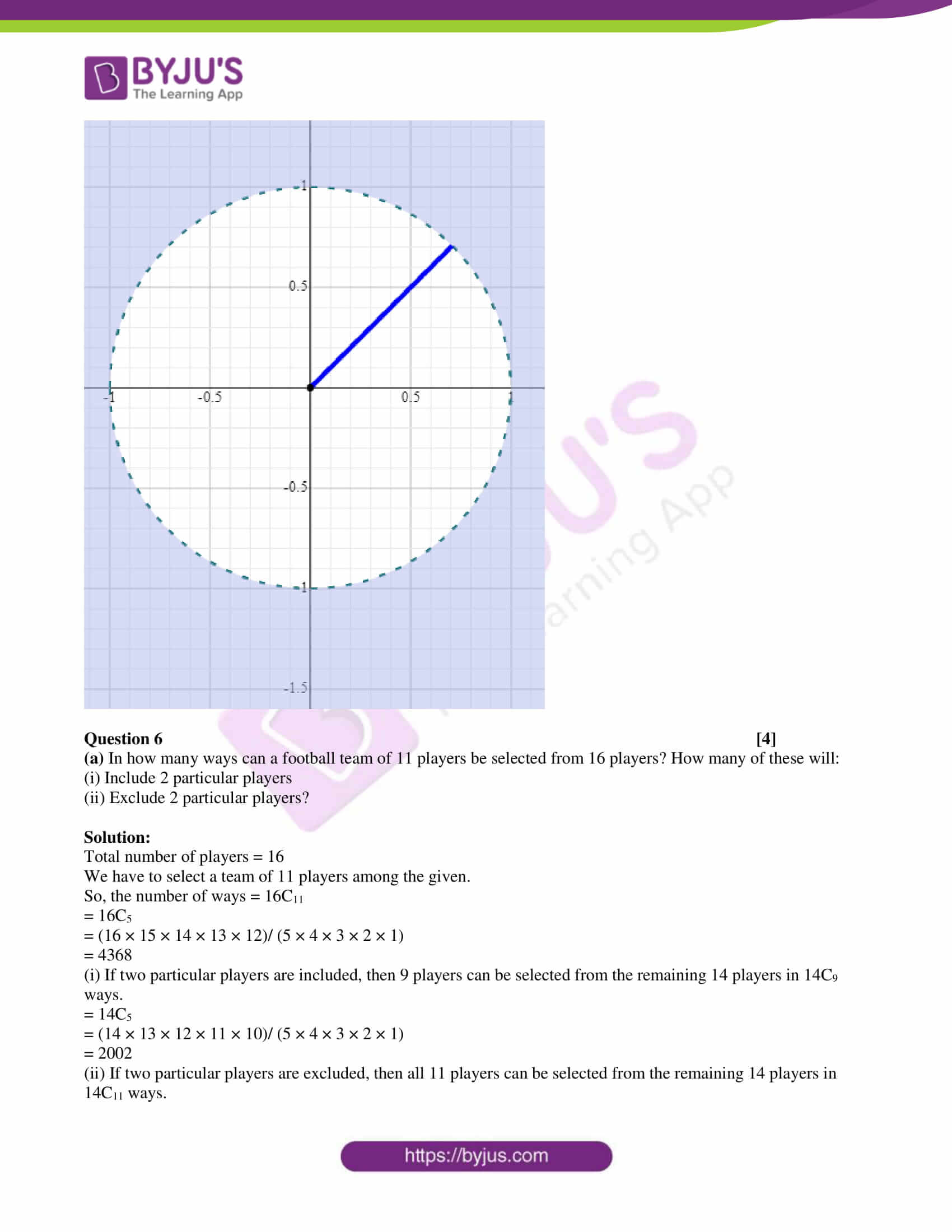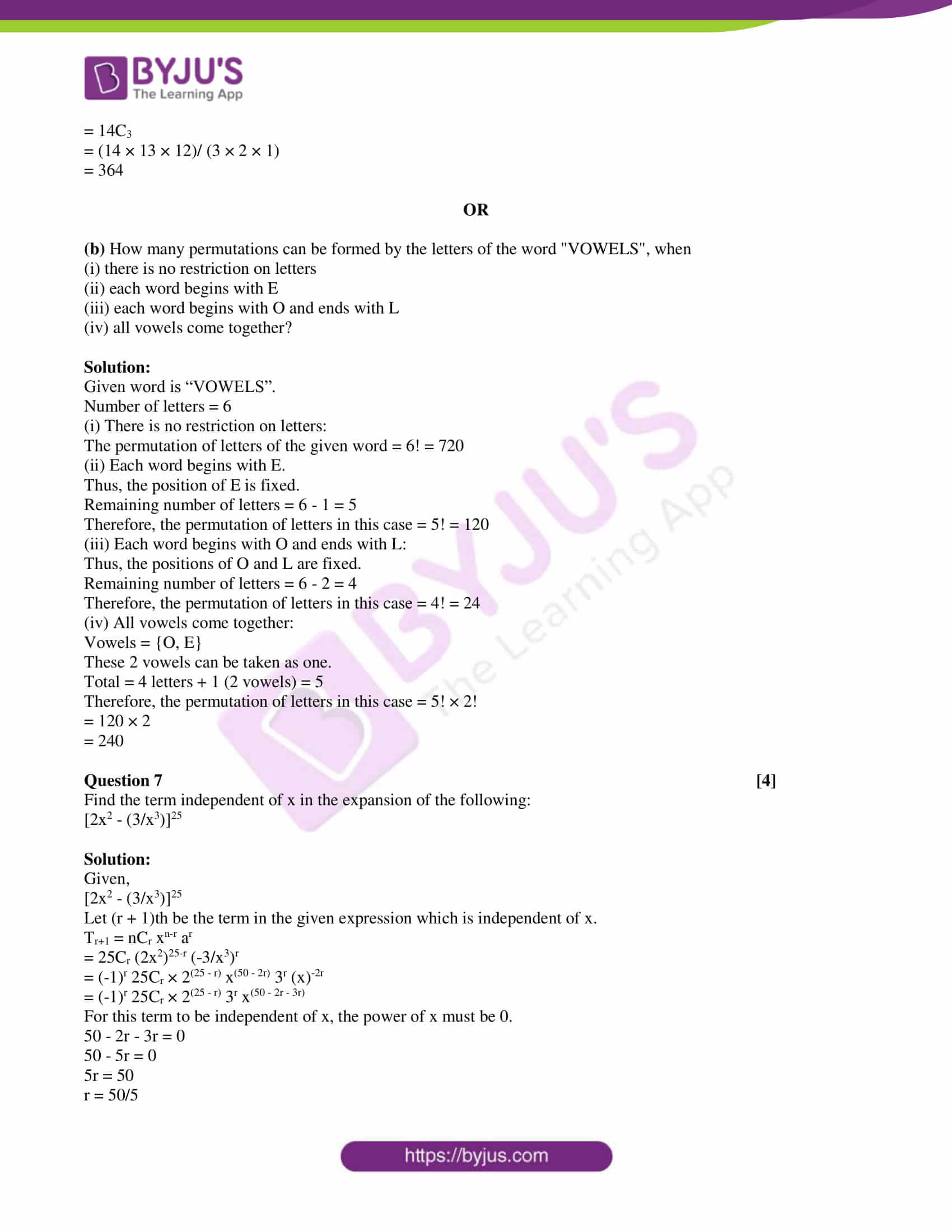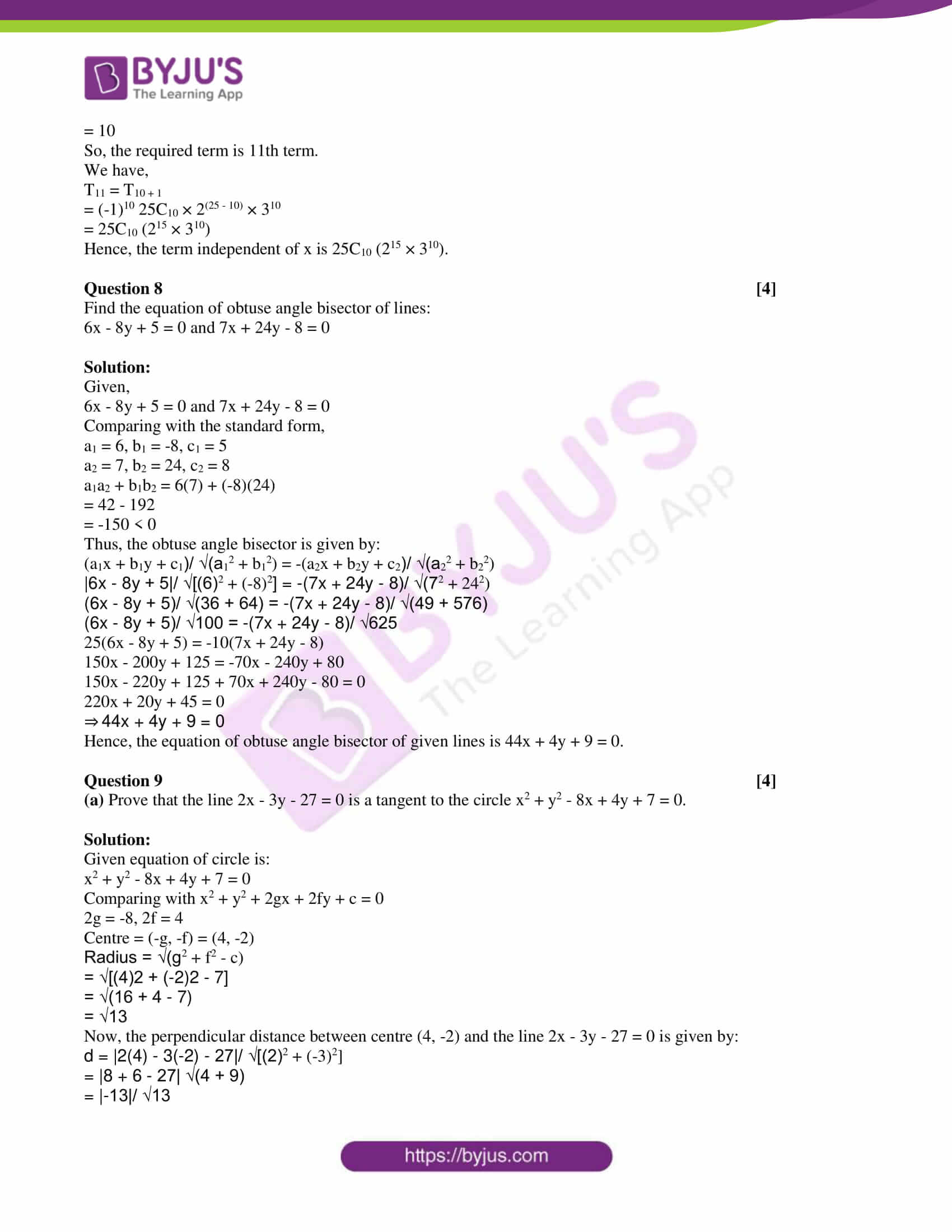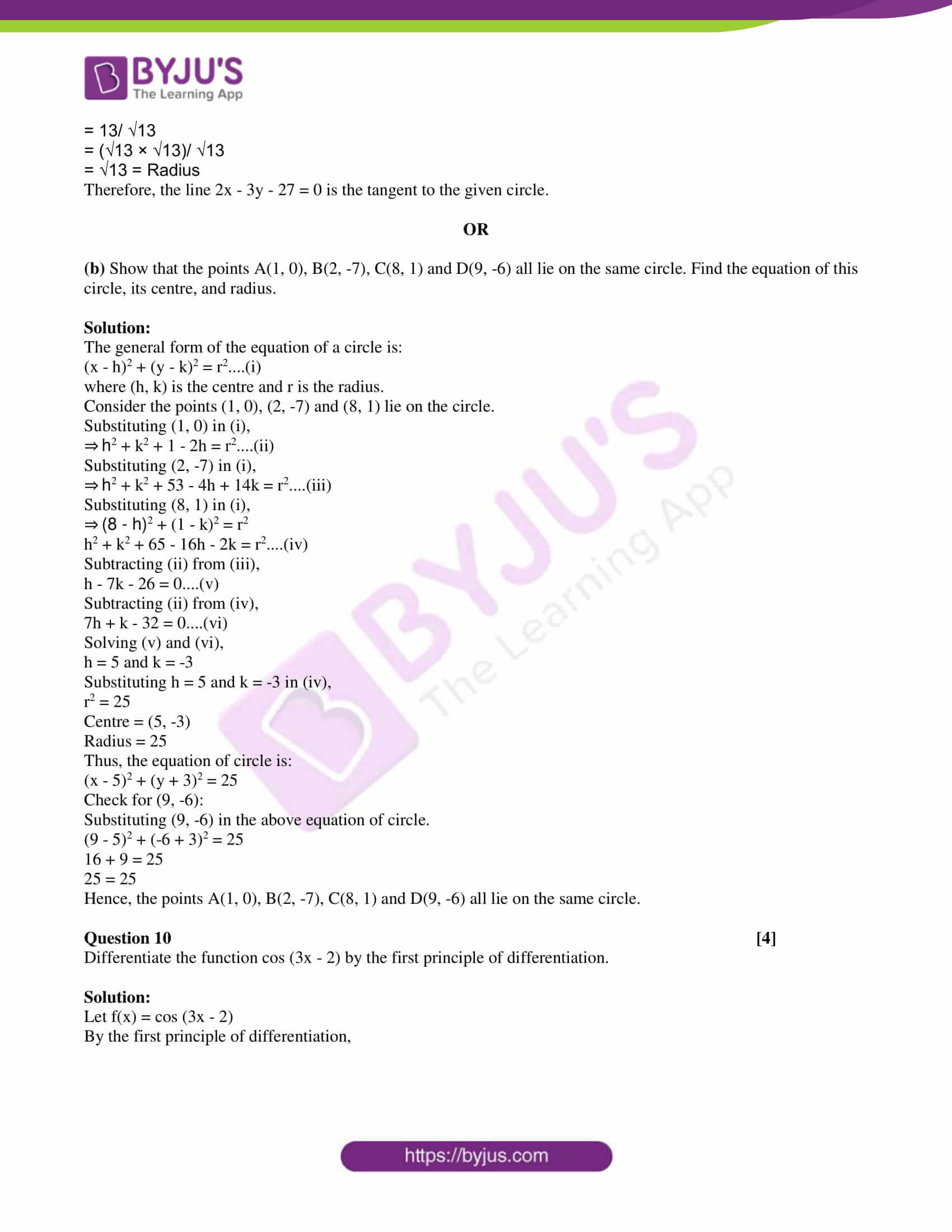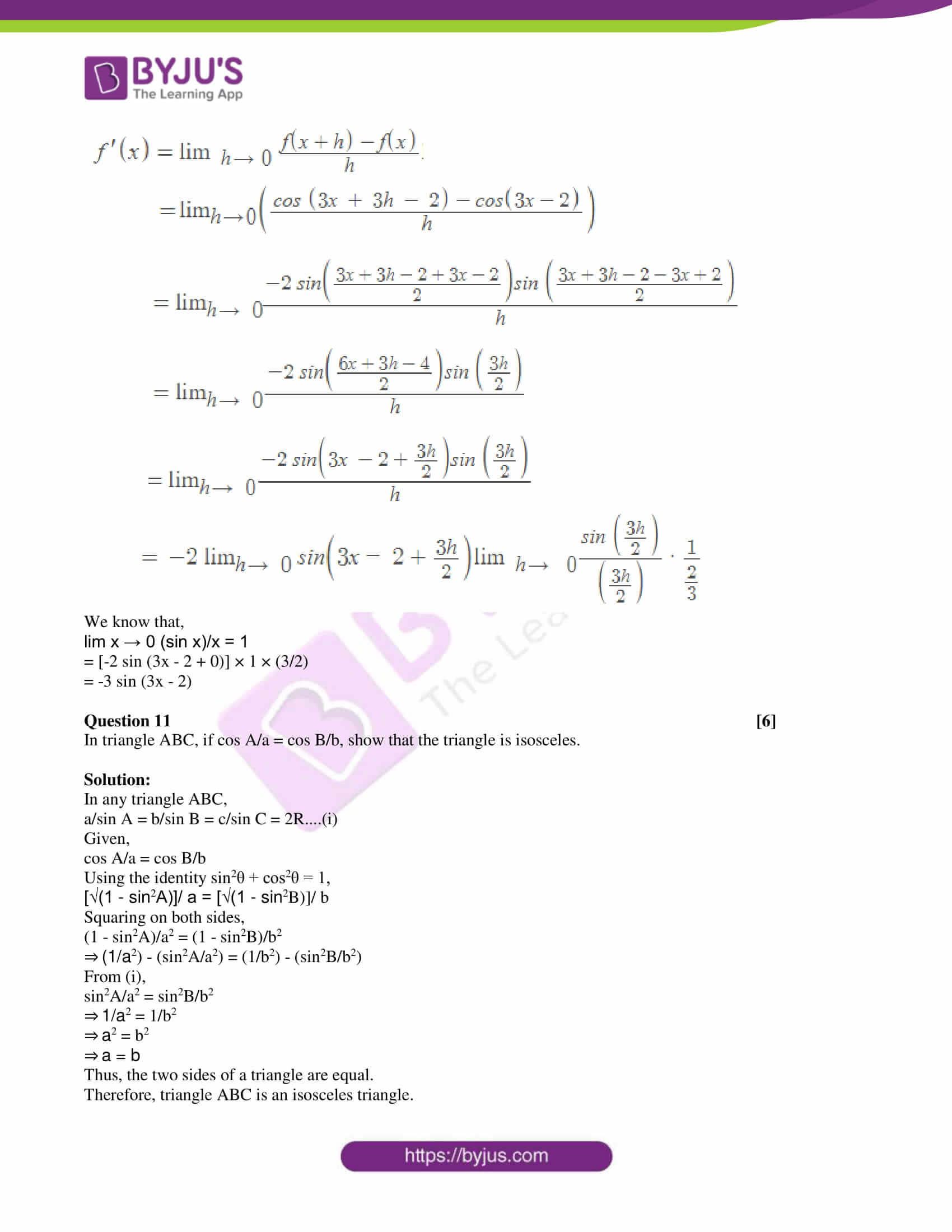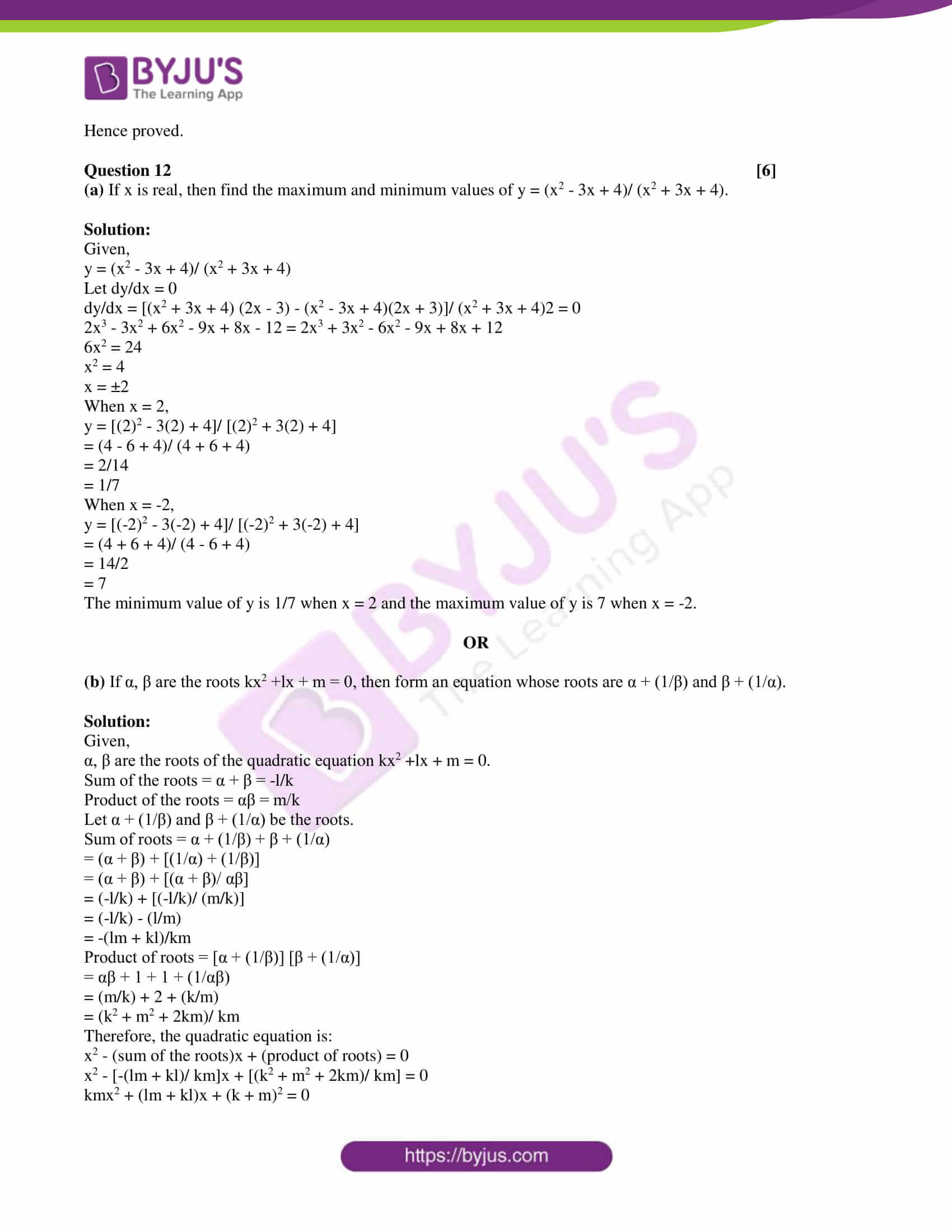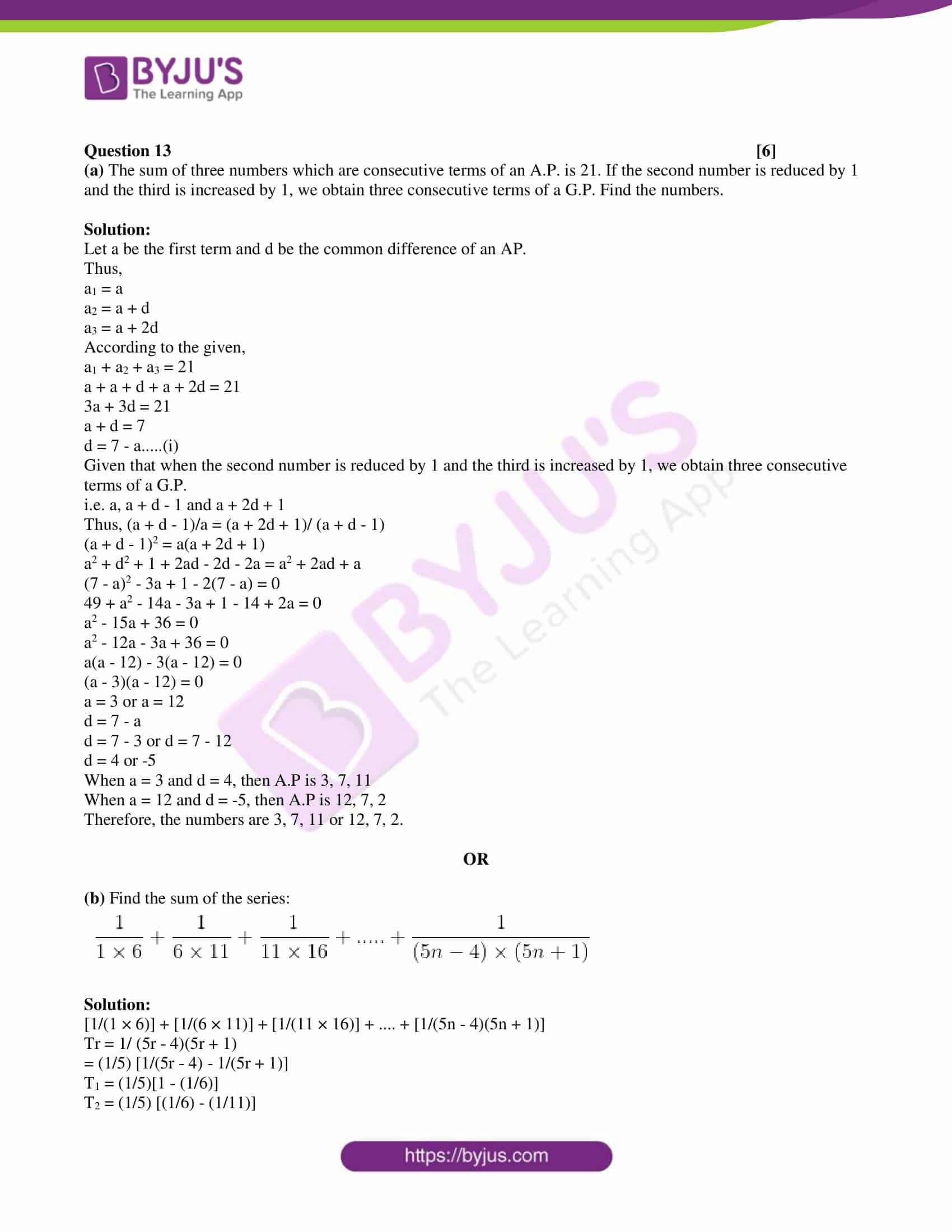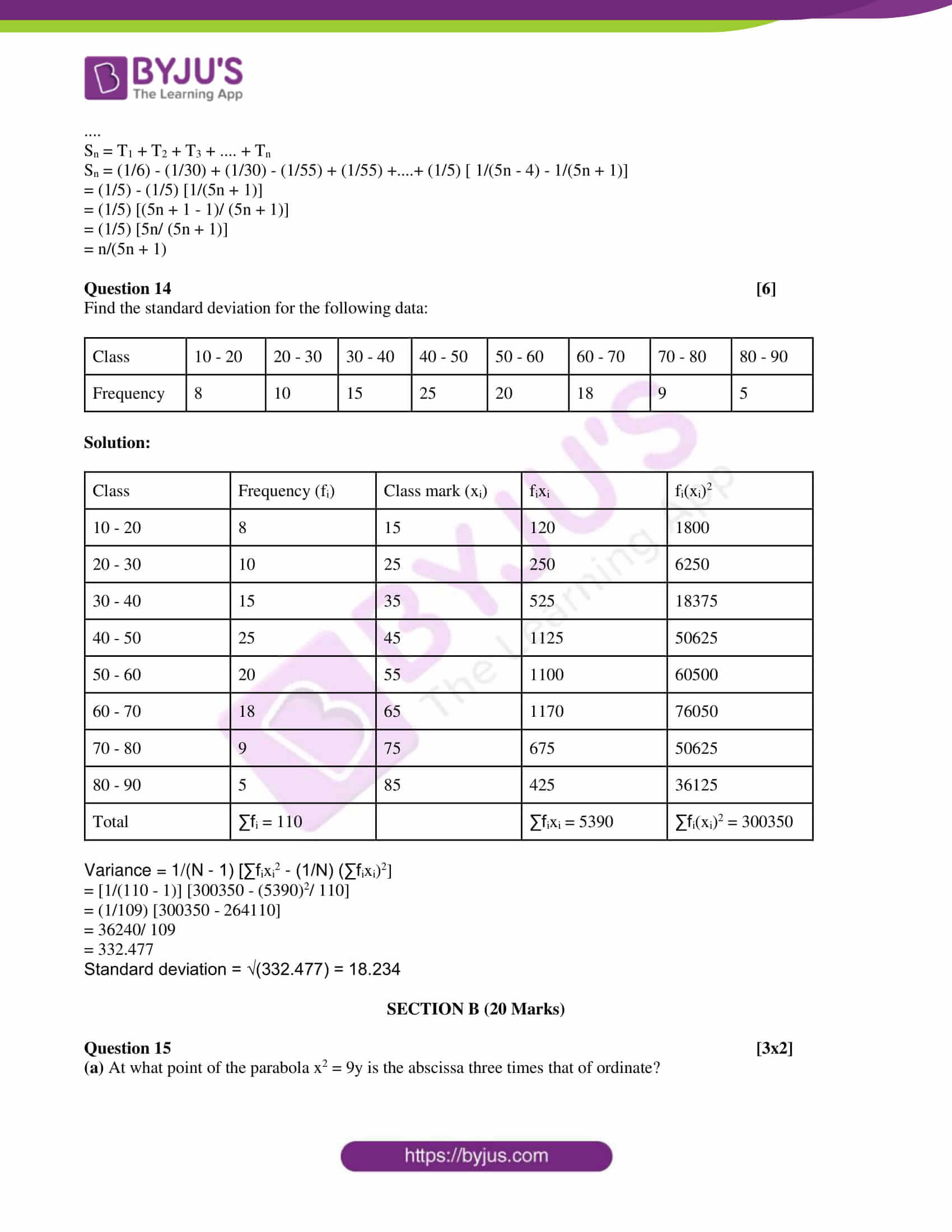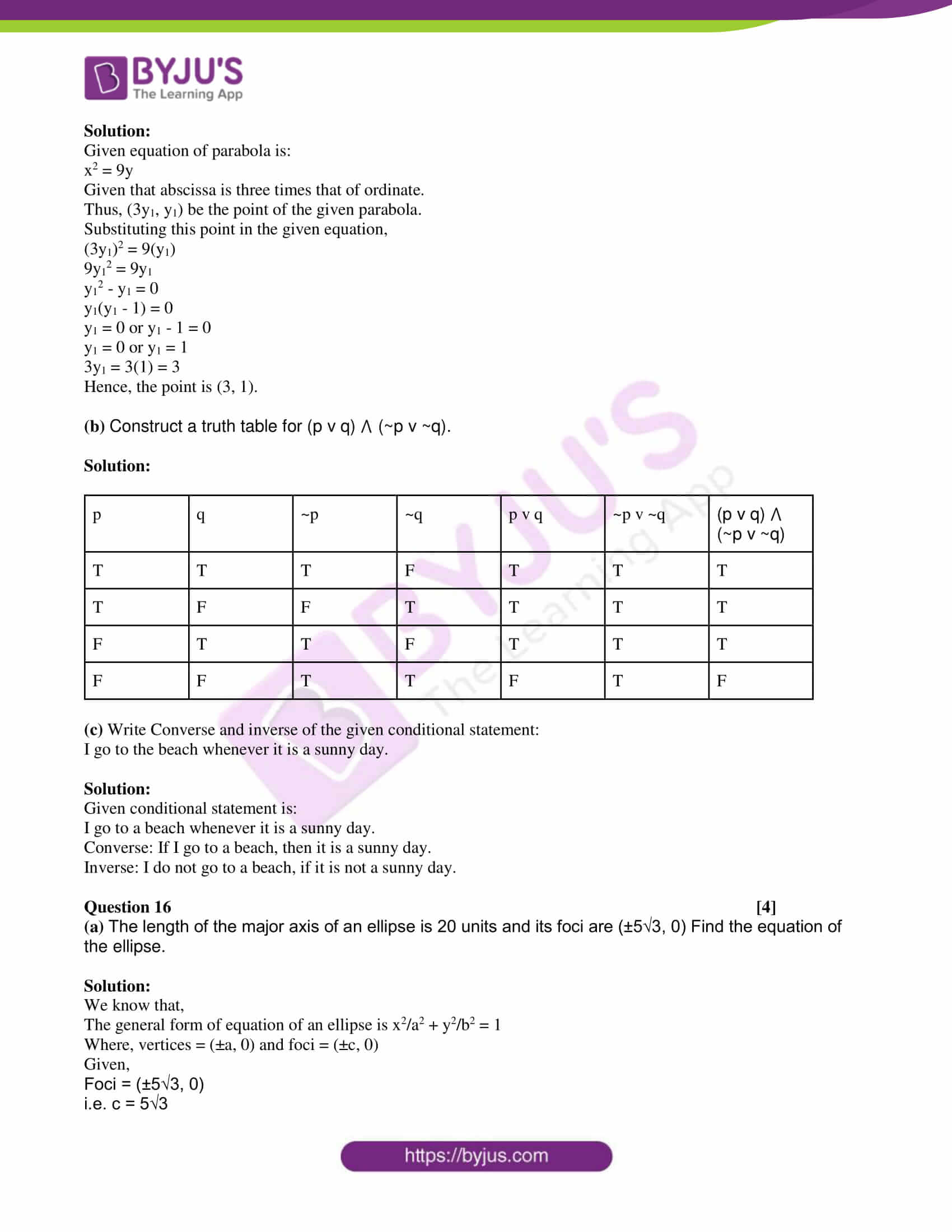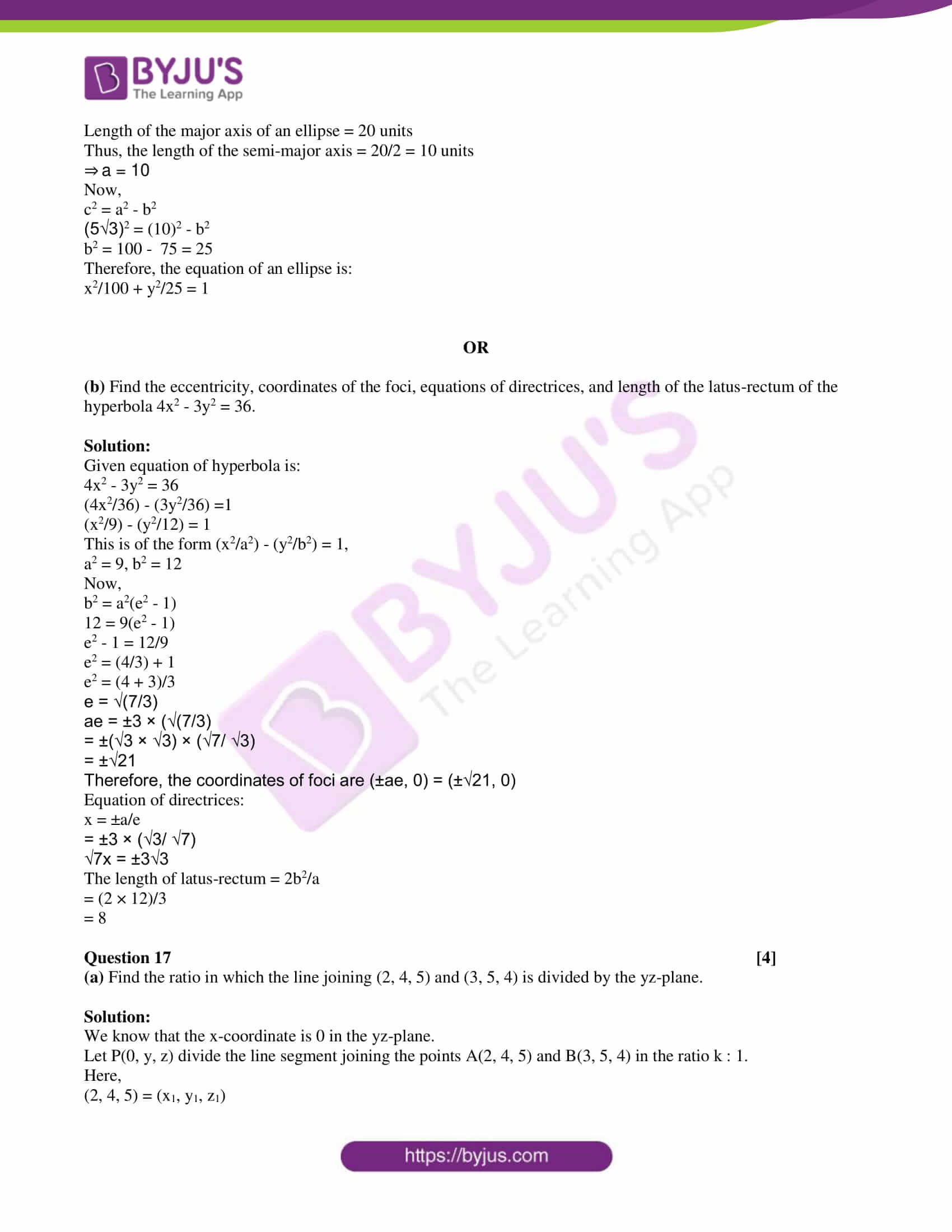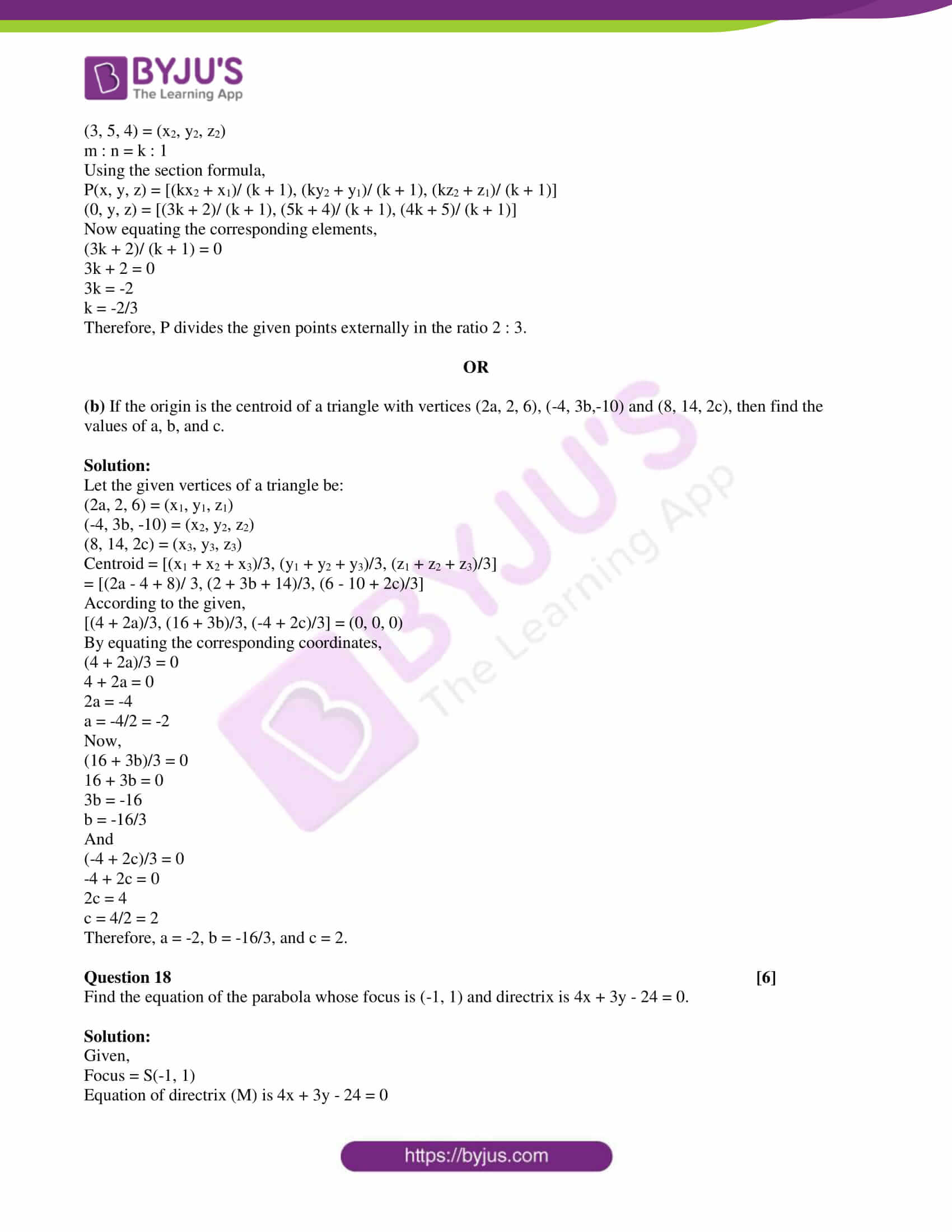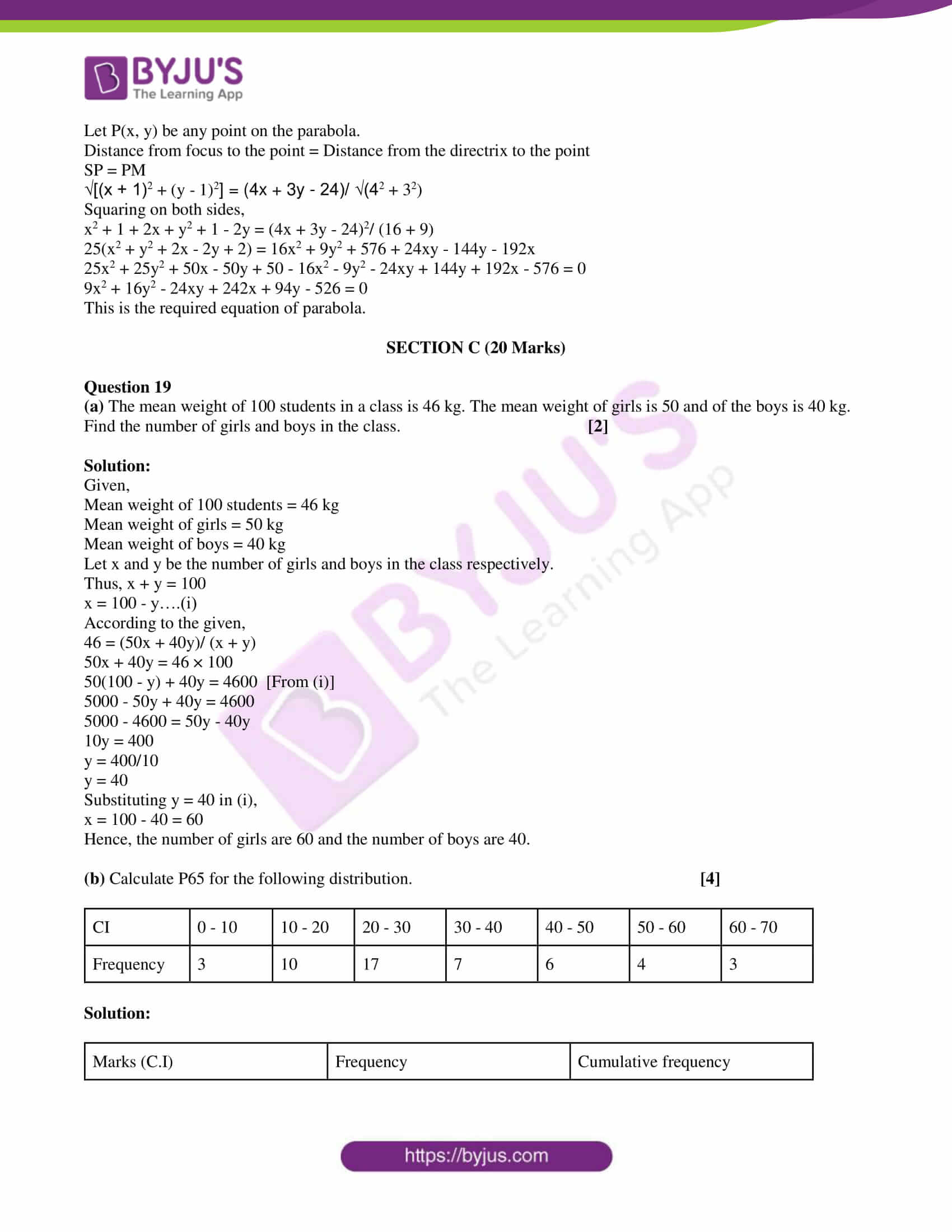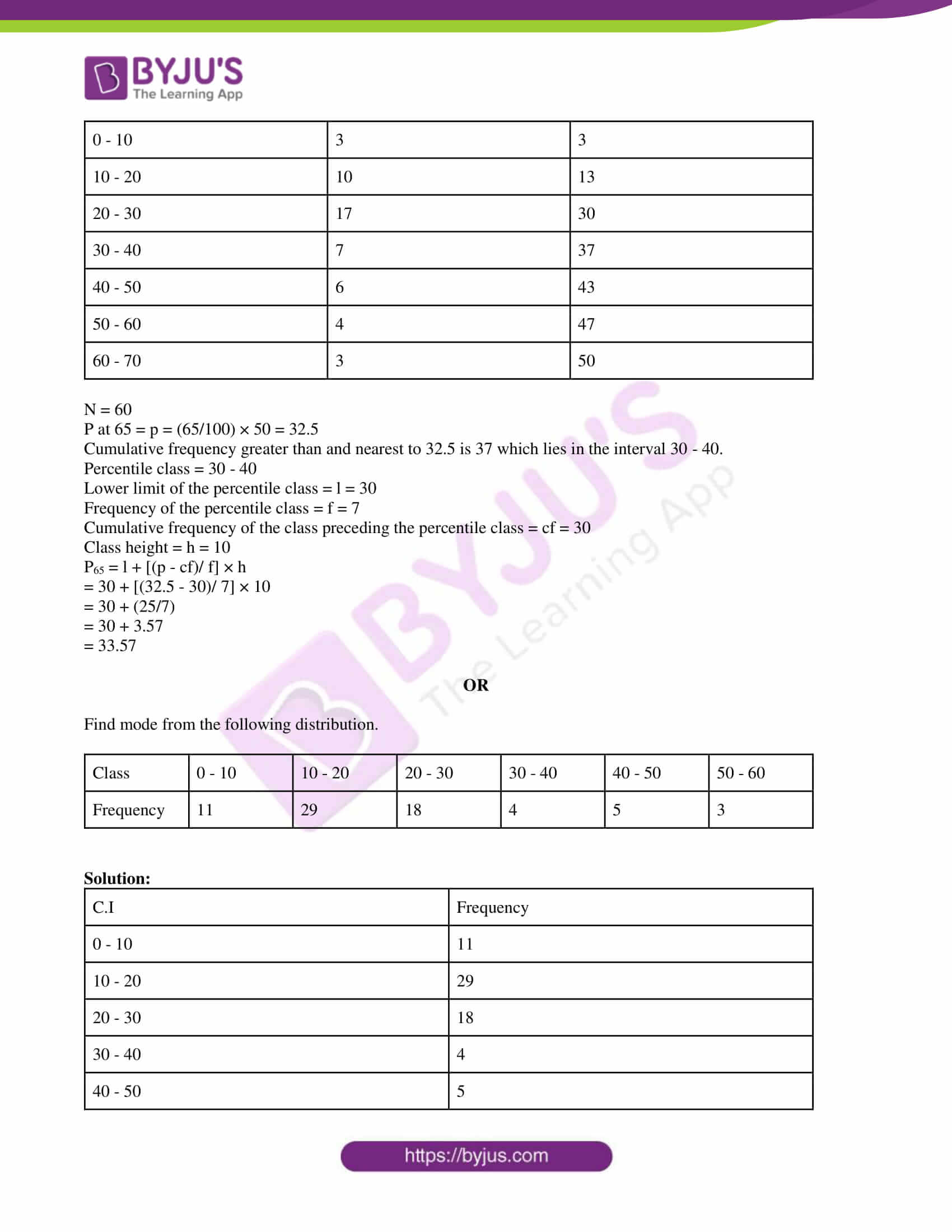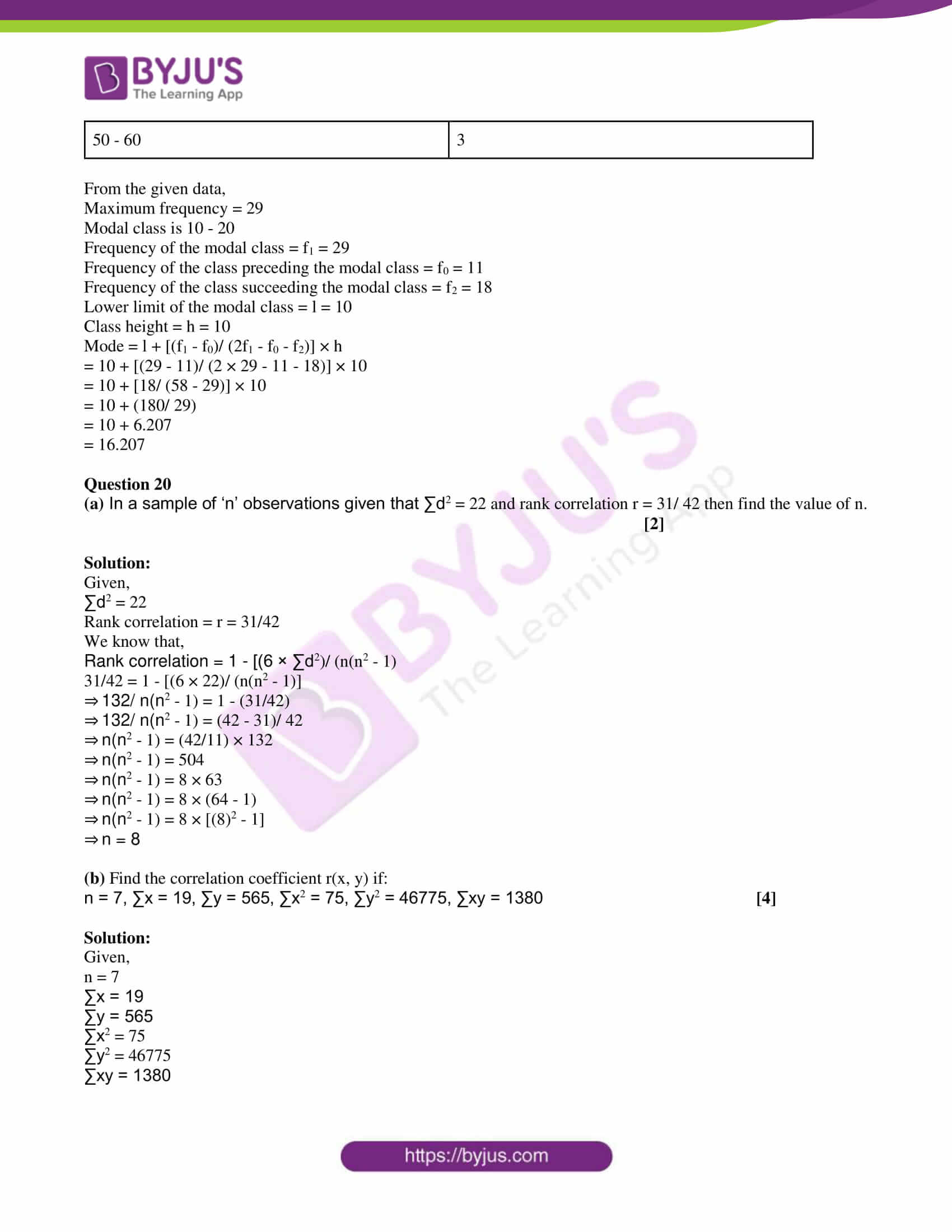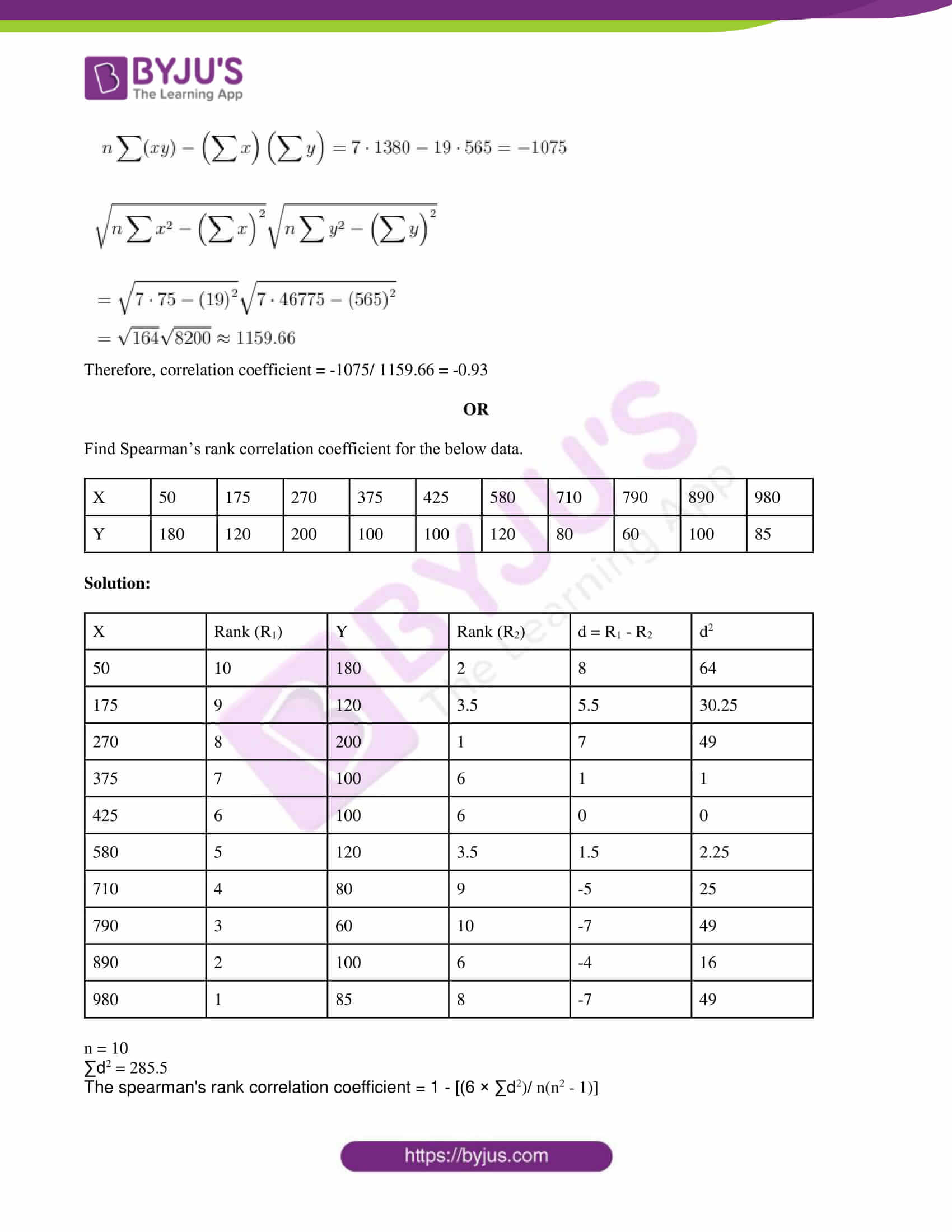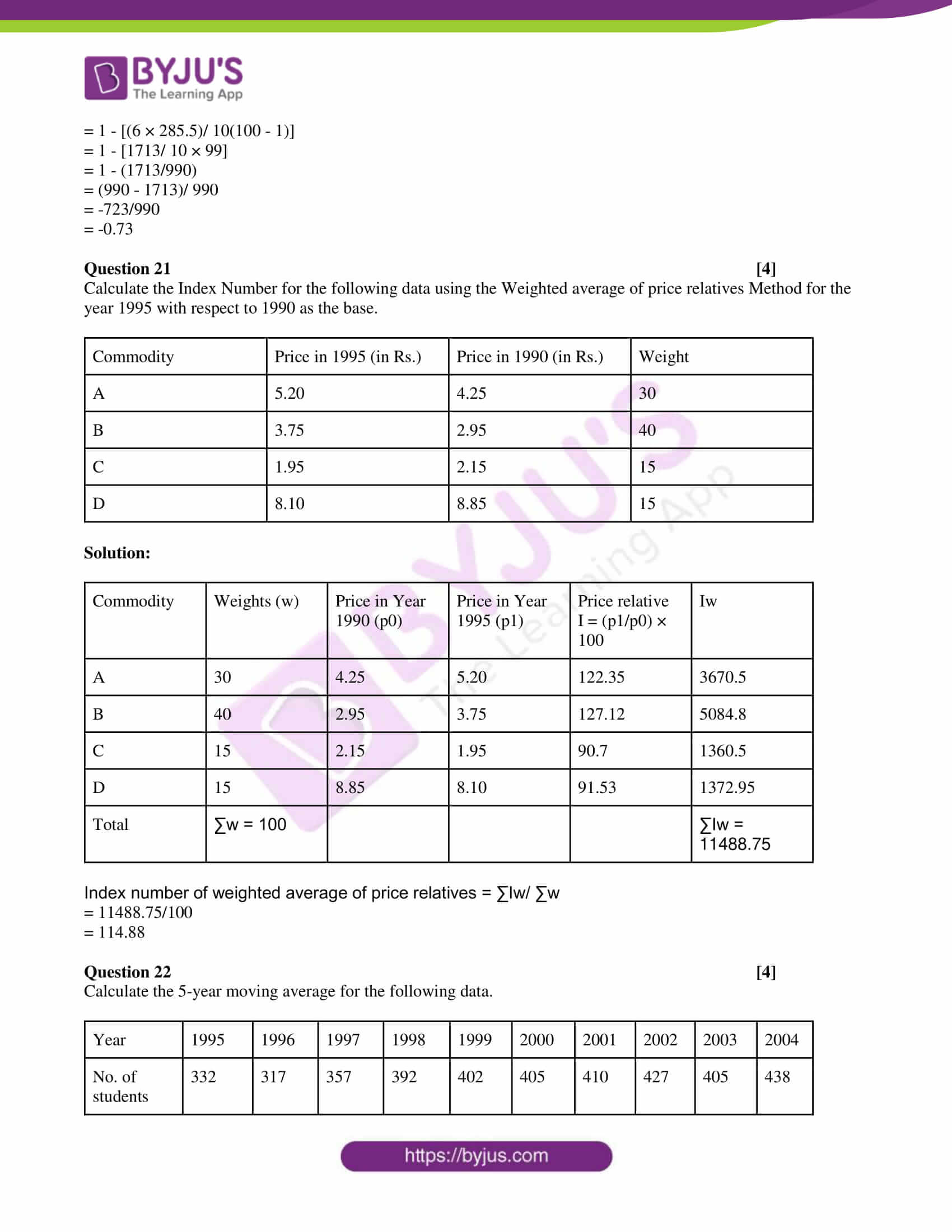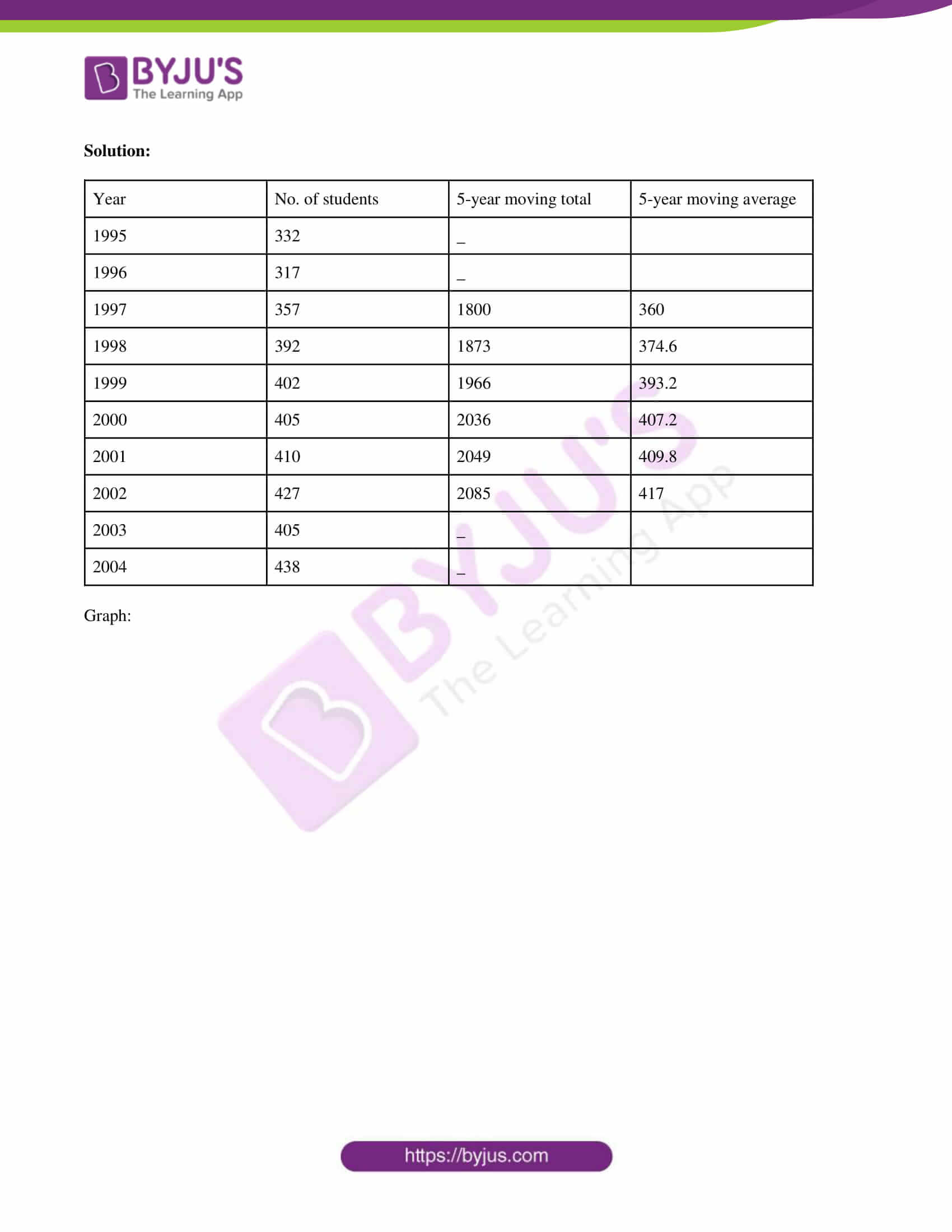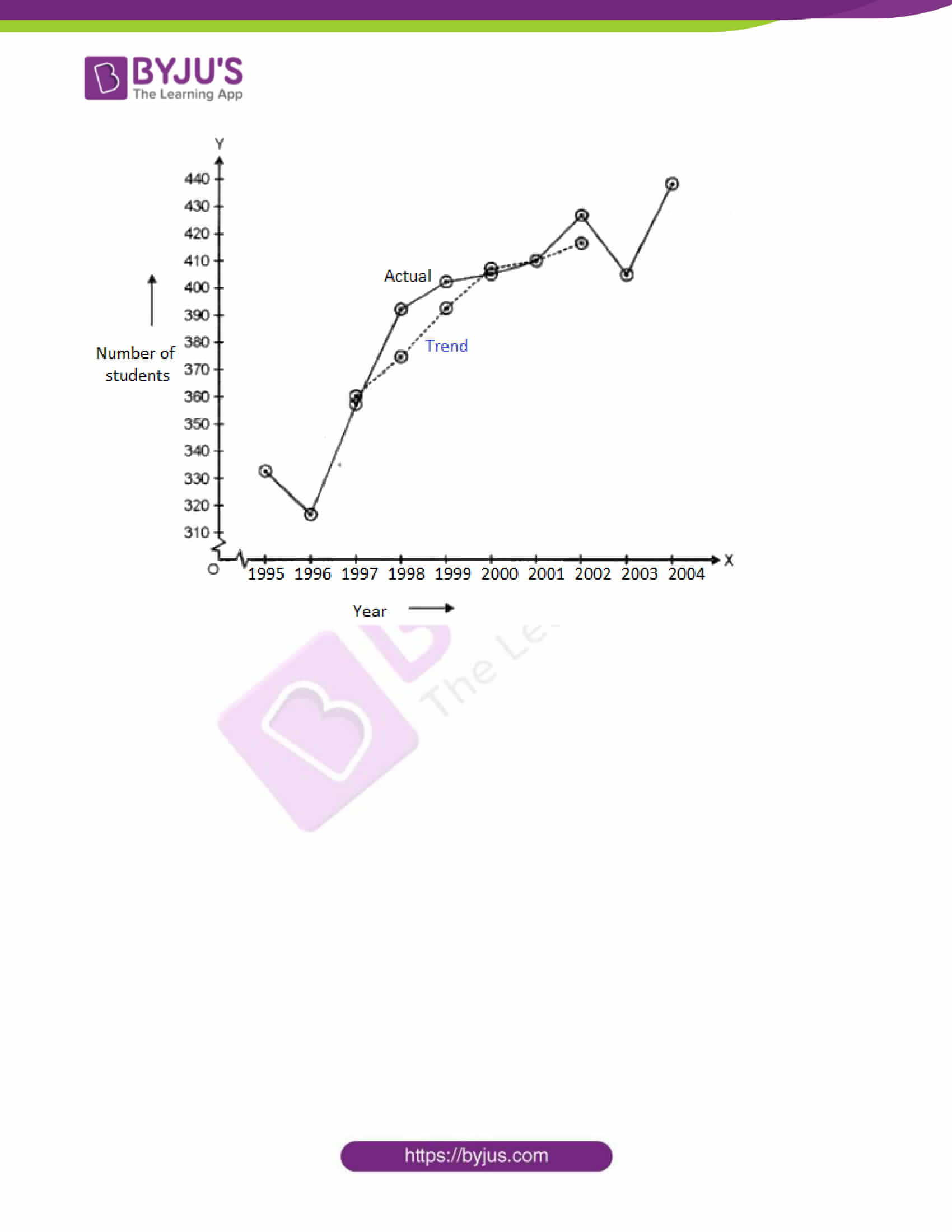SECTION – A

Question 1 [10×2]

(i) Let f : R → R be defined as f(x) = x2 + 1, find f-1(-7) = x.

Solution:

We know that,

If f : A → B such that y ∈ B, then f-(y) = {x ∈ A : f(x) = y}

Given,

f(x) = x2 + 1

f-1(-7) = {x ∈ R ; f(x) = -7}

Now,

x2 + 1 = -7

x2 = -7 – 1

x2 = -8

This is not possible.

Therefore, f-1(-7) = Φ

(ii) If a ∈ {2, 4, 6, 9} and b ∈ {4, 6, 18, 27}, then form the set of all ordered pairs (a, b) such that a divides b and a < b.

Solution:

Given,

a ∈ {2, 4, 6, 9} and b ∈{4, 6, 18, 27}

Now,

2 divides 4, 6, 18 and is also 2 < 4, 6, 8

4 divides 4 but both are equal

6 divides 6, 18 and 6 is less than 18

9 divides 18, 27 and is less than 18 and 27

Let us form ordered pairs (a, b) such that a divides b and a < b.

Hence, the required ordered pairs of (a, b) are {(2, 4), (2, 6), (2, 18), (6, 18), (9, 18) and (9, 27)}

(iii) Prove that cos x + cos (120° – x) + cos (120° + x) = 0.

Solution:

LHS = cos x + cos (120° – x) + cos (120° + x)

= cos x + (cos 120° cos x + sin 120° sin x) + (cos 120° cos x – sin 120° sin x)

= cos x + 2 cos 120° cos x

= cos x + 2 cos x cos (90° + 30°)

= cos x + 2 cos x (- sin 30°)

= cos x + 2 × (1/2) cos x

= cos x – cos x

= 0

= RHS

Hence proved.

(iv) Express (1 + i)3 – (1 – i)3 in the form of a + ib, find the value of a and b.

Solution:

(1 + i)3 – (1 – i)3

= (1)3 + 3(1)2(i) + 3(1)(i)2 + (i)3 – [(1)3 – 3(1)2(i) + 3(1)(i)2 – (i)3]

= 1 + 3i + 3i2 + i3 – (1 – 3i + 3i2 – i3)

= 1 + 3i + 3i2 + i3 – 1 + 3i – 3i2 + i3

= 6i + 2i3

= 6i + 2i(i2)

= 6i + 2i(-1)

= 6i – 2i

= 4i

= 0 + 4i

This is of the form a + ib.

Therefore, a = 0 and b = 4.

(v) Prove that b sin B – c sin C = a sin (B – c).

Solution:

By sine rule in a triangle,

a/sin A = b/sin B = c/sin C = k

⇒ a = k sin A, b = k sin B, c = k sin C…..(i)

LHS = b sin B – c sin C

k sin B sin B – k sin C sin C [From (i)]

= k (sin2B – sin2C)

= k [sin (B + c) sin (B – C)]….(ii)

We know that,

A + B + C = π (by the angle sum property of a triangle)

B + C = π – A….(iii)

From (ii) and (iii),

= k [sin (π – A) sin (B – C)]

= k [sin A sin (B – C)]

= (k sin A) sin (B – C)

= a sin (B – C) [again from (i)]

= RHS

Hence proved.

(vi) If α and β are the roots of the equation px2 – qx + r = 0, find α3 + β3.

Solution:

Given,

α and β are the roots of the equation px2 – qx + r = 0.

Sum of the roots = α + β = -(-q)/p – q/p

Product of the roots = αβ = r/p

α3 + β3 = (α + β) (α2 + β2 – αβ)

= (q/p) [α2 + β2 + 2αβ – 3αβ]

= (q/p) [(α + β)2 – 3αβ]

= (q/p) [(q/p)2 – 3(r/p)]

= (q/p) [(q2/p2) – (3r/p)]

= (q/p) [(q2 – 3pr)/ p2]

= (q3 – 3pqr)/ p3

(vii) In how many ways can 10 members of a committee sit around a round table so that the secretary and the joint secretary are always as the neighbors of the present?

Solution:

Let us assume the President, Secretary, and Joint secretary be 1 member.

Remaining = 10 – 3 = 7

Now we consider the total members as 8 (i.e. 7 members and 1 member that represents three specified people).

The number of ways of arranging these 8 members around a table = (8 – 1)! = 7!

And, the number of ways of arranging three specified people such that Secretary and the Joint secretary be the neighbors of the President = 2

Hence, the total number of arrangements = 7! × 2

= 5040 × 2

= 10080

(viii) In a single throw of three dice, find the probability of getting a total of 17 or 18.

Solution:

Given that three dice are thrown simultaneously.

Total number of possible outcomes = 63 = 216

i.e. n (S) = 216

Let E be the event of getting a total of 17 or 18.

E = {(6, 6, 5) (6, 5, 6) (5, 6, 6) (6, 6, 6)}

n (E) = 4

P (E) = n (E) / n (S)

= 4 / 216

= 1/54

(ix) Evaluate: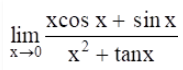Solution: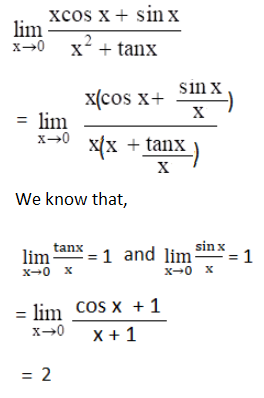(x) Differentiate: f(x) = (x2 + 1)/ (x + 1)

Solution:

Given,

f(x) = (x2 + 1)/ (x + 1)

Let u = x2 + 1 and v = x + 1

⇒ du/dx = d/dx (x2 + 1) = 2x

And

dv/dx = d/dx (x + 1) = 1

We know that,

d/dx (u/v) = [v(du/dx) – u(dv/dx)] / v2

= [(x + 1)(2x) – (x2 + 1)(1)]/ (x + 1)2

= [2x2 + 2x – (x2 + 1)]/ (x + 1)2

= (2x2 + 2x – x2 – 1)/ (x + 1)2

= (x2 + 2x – 1)/ (x + 1)2

Therefore, the derivative of a given f(x) is (x2 + 2x – 1)/ (x + 1)2.

Question 2 

Find the domain and range of the function f(x) = 1/ √(2x – 3).

Solution:

f(x) = 1/ √(2x – 3)

Let 1/ √(2x – 3) = y….(i)

The given function will be defined only if 2x – 3 > 0

⇒ 2x > 3

⇒ x > 3/2

Thus, the function is defined for all the real values greater than 3/2.

From (i),

1/√(2x – 3) = y

Squaring on both sides,

1/(2x – 3) = y2

⇒ 2x – 3 = 1/y2

⇒ 2x = (1/y2) + 3

⇒ x = (1 + 3y2)/ 2y2

This can be defined when y > 0.

Therefore, the domain of f(x) is (3/2, ∞) and the range is (0, ∞).

Question 3 

(a) Solve for x: cos x + sin x = cos 2x + sin 2x

Solution:

cos x + sin x = cos 2x + sin 2x

cox – cos 2x = sin 2x – sin x

-2 [sin (2x + x)/2] [sin (2x – x)/2] = 2 [cos (2x + x)/2] [sin (2x – x)/2]

2 sin (3x/2) sin (x/2) = 2 cos (3x/2) sin (x/2)

sin (3x/2) sin (x/2) – cos (3x/2) sin (x/2) = 0

sin (x/2) [sin (3x/2) – cos (3x/2)] = 0

sin (x/2) = 0 or sin (3x/2) = cos (3x/2)

sin (x/2) = sin mπ or sin (3x/2) / cos (3x/2) = 0

sin (x/2) = sin mπ or tan (3x/2) = 1

sin (x/2) = sin mπ or tan (3x/2) = tan π/4

x/2 = mπ or 3x/2 = nπ + π/4

x = 2mπ or x = 2nπ/3 + π/6

Hence, the general solution is

x = 2mπ or 2nπ/3 + π/6, where m, n ϵ Z.

OR

(b) If A + B + C = π, prove that tan 2A + tan 2B + tan 2C = tan 2A tan 2B tan 2C.

Solution:

Given,

A + B + C = π

A + B = π – C

Multiplying by 2 on both sides,

2A + 2B = 2π – 2C

Taking “tangent” on both sides,

tan (2A + 2B) = tan (2π – 2C)

(tan 2A + tan 2B)/ (1 – tan 2A tan 2B) = – tan 2C

tan 2A + tan 2B = -tan 2C (1 – tan 2A tan 2B)

tan 2A + tan 2B = -tan 2C + tan 2A tan 2B tan 2C

tan 2A + tan 2B + tan 2C = tan 2A tan 2B tan 2C

Hence proved.

Question 4 

Prove that 32n + 2 – 8n – 9 is divisible by 8 for all n ϵ N.

Solution:

Let P(n) = 32n + 2 – 8n – 9 is divisible by 8

Let us check for n = 1,

P(1) = 3(2.1 + 2) – 8(1) – 9

= 34 – 8 – 9

= 81 – 17

= 64

= 8 (8), which is divisible by 8.

P(n) is true for n = 1.

Now, let us check for P(n) is true for n = k, and have to prove that P(k + 1) is also true.

P(k): 3(2k + 2) – 8k – 9 is divisible by 8

= 3(2k + 2) – 8k – 9 = 8M … (i)

We have to prove,

3(2k + 4) – 8(k + 1) – 9 is divisible by 8.

3[2(k + 1) + 2] – 8(k + 1) – 9

= 3(2k + 2) . 32 – 8k – 8 – 9

= (8M + 8k + 9)9 – 8k – 17 [From (i)]

= 72M + 72k + 81 – 8k – 17 using equation (1)

= 72M + 64k + 64

= 8(9M + 8k + 8)

= 8P

P(n) is also true for n = k + 1.

Hence, P(n) is true for all n ∈ N.

Question 5 

Find the locus of z satisfying the inequality |(z + 2i)/ (2z + 1)| < 1, where z = x + iy. Represent it in the argand plane.

Solution:

Given,

z = x + iy

|(z + 2i)/ (2z + 1)| < 1

|(z + 2i)/ (2z + i)| < 1

⇒ |z + 2i|/ |2z + i| < 1

⇒ |z + 2i| < |2z + i|

⇒ |x + iy + 2i| < |2(x + iy) + i|

⇒ |x + (y + 2)i| < |2x + (2y + 1)i|

⇒ √[x2 + (y + 2)2] < √[(2x)2 + (2y + 1)2]

Squaring on both sides,

⇒ x2 + y2 + 4y + 4 < 4x2 + 4y2 + 1 + 4y

⇒ y2 + 4y + 4 < 3x2 + 4y2 + 4y + 1

⇒ 3x2 + 4y2 – y2 > 4 – 1

⇒ 3x2 + 3y2 > 3

⇒ x2 + y2 > 1

Thus, the locus of z represents the circle with centre (0, 0) and radius 1 unit.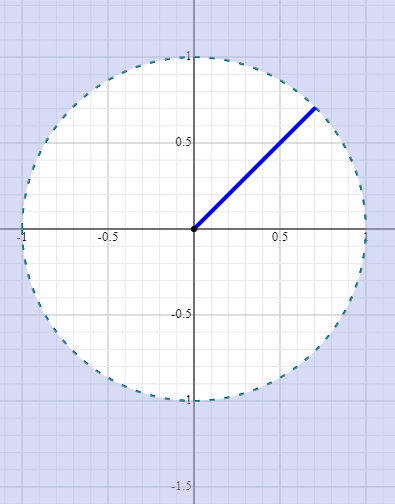Question 6 

(a) In how many ways can a football team of 11 players be selected from 16 players? How many of these will:

(i) Include 2 particular players

(ii) Exclude 2 particular players?

Solution:

Total number of players = 16

We have to select a team of 11 players among the given.

So, the number of ways = 16C11

= 16C5

= (16 × 15 × 14 × 13 × 12)/ (5 × 4 × 3 × 2 × 1)

= 4368

(i) If two particular players are included, then 9 players can be selected from the remaining 14 players in 14C9 ways.

= 14C5

= (14 × 13 × 12 × 11 × 10)/ (5 × 4 × 3 × 2 × 1)

= 2002

(ii) If two particular players are excluded, then all 11 players can be selected from the remaining 14 players in 14C11 ways.

= 14C3

= (14 × 13 × 12)/ (3 × 2 × 1)

= 364

OR

(b) How many permutations can be formed by the letters of the word “VOWELS”, when

(i) there is no restriction on letters

(ii) each word begins with E

(iii) each word begins with O and ends with L

(iv) all vowels come together?

Solution:

Given word is “VOWELS”.

Number of letters = 6

(i) There is no restriction on letters:

The permutation of letters of the given word = 6! = 720

(ii) Each word begins with E.

Thus, the position of E is fixed.

Remaining number of letters = 6 – 1 = 5

Therefore, the permutation of letters in this case = 5! = 120

(iii) Each word begins with O and ends with L:

Thus, the positions of O and L are fixed.

Remaining number of letters = 6 – 2 = 4

Therefore, the permutation of letters in this case = 4! = 24

(iv) All vowels come together:

Vowels = {O, E}

These 2 vowels can be taken as one.

Total = 4 letters + 1 (2 vowels) = 5

Therefore, the permutation of letters in this case = 5! × 2!

= 120 × 2

= 240

Question 7 

Find the term independent of x in the expansion of the following:

[2x2 – (3/x3)]25

Solution:

Given,

[2x2 – (3/x3)]25

Let (r + 1)th be the term in the given expression which is independent of x.

Tr+1 = nCr xn-r ar

= 25Cr (2x2)25-r (-3/x3)r

= (-1)r 25Cr × 2(25 – r) x(50 – 2r) 3r (x)-2r

= (-1)r 25Cr × 2(25 – r) 3r x(50 – 2r – 3r)

For this term to be independent of x, the power of x must be 0.

50 – 2r – 3r = 0

50 – 5r = 0

5r = 50

r = 50/5

= 10

So, the required term is 11th term.

We have,

T11 = T10 + 1

= (-1)10 25C10 × 2(25 – 10) × 310

= 25C10 (215 × 310)

Hence, the term independent of x is 25C10 (215 × 310).

Question 8 

Find the equation of obtuse angle bisector of lines:

6x – 8y + 5 = 0 and 7x + 24y – 8 = 0

Solution:

Given,

6x – 8y + 5 = 0 and 7x + 24y – 8 = 0

Comparing with the standard form,

a1 = 6, b1 = -8, c1 = 5

a2 = 7, b2 = 24, c2 = 8

a1a2 + b1b2 = 6(7) + (-8)(24)

= 42 – 192

= -150 < 0

Thus, the obtuse angle bisector is given by:

(a1x + b1y + c1)/ √(a12 + b12) = -(a2x + b2y + c2)/ √(a22 + b22)

|6x – 8y + 5|/ √[(6)2 + (-8)2] = -(7x + 24y – 8)/ √(72 + 242)

(6x – 8y + 5)/ √(36 + 64) = -(7x + 24y – 8)/ √(49 + 576)

(6x – 8y + 5)/ √100 = -(7x + 24y – 8)/ √625

25(6x – 8y + 5) = -10(7x + 24y – 8)

150x – 200y + 125 = -70x – 240y + 80

150x – 220y + 125 + 70x + 240y – 80 = 0

220x + 20y + 45 = 0

⇒ 44x + 4y + 9 = 0

Hence, the equation of obtuse angle bisector of given lines is 44x + 4y + 9 = 0.

Question 9 

(a) Prove that the line 2x – 3y – 27 = 0 is a tangent to the circle x2 + y2 – 8x + 4y + 7 = 0.

Solution:

Given equation of circle is:

x2 + y2 – 8x + 4y + 7 = 0

Comparing with x2 + y2 + 2gx + 2fy + c = 0

2g = -8, 2f = 4

Centre = (-g, -f) = (4, -2)

Radius = √(g2 + f2 – c)

= √[(4)2 + (-2)2 – 7]

= √(16 + 4 – 7)

= √13

Now, the perpendicular distance between centre (4, -2) and the line 2x – 3y – 27 = 0 is given by:

d = |2(4) – 3(-2) – 27|/ √[(2)2 + (-3)2]

= |8 + 6 – 27| √(4 + 9)

= |-13|/ √13

= 13/ √13

= (√13 × √13)/ √13

Therefore, the line 2x – 3y – 27 = 0 is the tangent to the given circle.

OR

(b) Show that the points A(1, 0), B(2, -7), C(8, 1) and D(9, -6) all lie on the same circle. Find the equation of this circle, its centre, and radius.

Solution:

The general form of the equation of a circle is:

(x – h)2 + (y – k)2 = r2….(i)

where (h, k) is the centre and r is the radius.

Consider the points (1, 0), (2, -7) and (8, 1) lie on the circle.

Substituting (1, 0) in (i),

⇒ h2 + k2 + 1 – 2h = r2….(ii)

Substituting (2, -7) in (i),

⇒ h2 + k2 + 53 – 4h + 14k = r2….(iii)

Substituting (8, 1) in (i),

⇒ (8 – h)2 + (1 – k)2 = r2

h2 + k2 + 65 – 16h – 2k = r2….(iv)

Subtracting (ii) from (iii),

h – 7k – 26 = 0….(v)

Subtracting (ii) from (iv),

7h + k – 32 = 0….(vi)

Solving (v) and (vi),

h = 5 and k = -3

Substituting h = 5 and k = -3 in (iv),

r2 = 25

Centre = (5, -3)

Thus, the equation of circle is:

(x – 5)2 + (y + 3)2 = 25

Check for (9, -6):

Substituting (9, -6) in the above equation of circle.

(9 – 5)2 + (-6 + 3)2 = 25

16 + 9 = 25

25 = 25

Hence, the points A(1, 0), B(2, -7), C(8, 1) and D(9, -6) all lie on the same circle.

Question 10 

Differentiate the function cos (3x – 2) by the first principle of differentiation.

Solution:

Let f(x) = cos (3x – 2)

By the first principle of differentiation,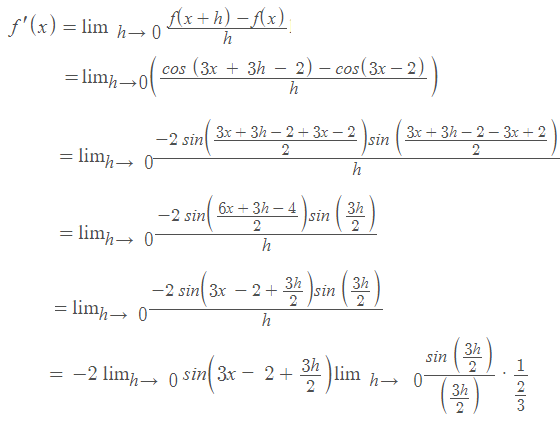We know that,

lim x → 0 (sin x)/x = 1

= [-2 sin (3x – 2 + 0)] × 1 × (3/2)

= -3 sin (3x – 2)

Question 11 

In triangle ABC, if cos A/a = cos B/b, show that the triangle is isosceles.

Solution:

In any triangle ABC,

a/sin A = b/sin B = c/sin C = 2R….(i)

Given,

cos A/a = cos B/b

Using the identity sin2θ + cos2θ = 1,

[√(1 – sin2A)]/ a = [√(1 – sin2B)]/ b

Squaring on both sides,

(1 – sin2A)/a2 = (1 – sin2B)/b2

⇒ (1/a2) – (sin2A/a2) = (1/b2) – (sin2B/b2)

From (i),

sin2A/a2 = sin2B/b2

⇒ 1/a2 = 1/b2

⇒ a2 = b2

⇒ a = b

Thus, the two sides of a triangle are equal.

Therefore, triangle ABC is an isosceles triangle.

Hence proved.

Question 12 

(a) If x is real, then find the maximum and minimum values of y = (x2 – 3x + 4)/ (x2 + 3x + 4).

Solution:

Given,

y = (x2 – 3x + 4)/ (x2 + 3x + 4)

Let dy/dx = 0

dy/dx = [(x2 + 3x + 4) (2x – 3) – (x2 – 3x + 4)(2x + 3)]/ (x2 + 3x + 4)2 = 0

2x3 – 3x2 + 6x2 – 9x + 8x – 12 = 2x3 + 3x2 – 6x2 – 9x + 8x + 12

6x2 = 24

x2 = 4

x = ±2

When x = 2,

y = [(2)2 – 3(2) + 4]/ [(2)2 + 3(2) + 4]

= (4 – 6 + 4)/ (4 + 6 + 4)

= 2/14

= 1/7

When x = -2,

y = [(-2)2 – 3(-2) + 4]/ [(-2)2 + 3(-2) + 4]

= (4 + 6 + 4)/ (4 – 6 + 4)

= 14/2

= 7

The minimum value of y is 1/7 when x = 2 and the maximum value of y is 7 when x = -2.

OR

(b) If α, β are the roots kx2 +lx + m = 0, then form an equation whose roots are α + (1/β) and β + (1/α).

Solution:

Given,

α, β are the roots of the quadratic equation kx2 +lx + m = 0.

Sum of the roots = α + β = -l/k

Product of the roots = αβ = m/k

Let α + (1/β) and β + (1/α) be the roots.

Sum of roots = α + (1/β) + β + (1/α)

= (α + β) + [(1/α) + (1/β)]

= (α + β) + [(α + β)/ αβ]

= (-l/k) + [(-l/k)/ (m/k)]

= (-l/k) – (l/m)

= -(lm + kl)/km

Product of roots = [α + (1/β)] [β + (1/α)]

= αβ + 1 + 1 + (1/αβ)

= (m/k) + 2 + (k/m)

= (k2 + m2 + 2km)/ km

x2 – (sum of the roots)x + (product of roots) = 0

x2 – [-(lm + kl)/ km]x + [(k2 + m2 + 2km)/ km] = 0

kmx2 + (lm + kl)x + (k + m)2 = 0

Question 13 

(a) The sum of three numbers which are consecutive terms of an A.P. is 21. If the second number is reduced by 1 and the third is increased by 1, we obtain three consecutive terms of a G.P. Find the numbers.

Solution:

Let a be the first term and d be the common difference of an AP.

Thus,

a1 = a

a2 = a + d

a3 = a + 2d

According to the given,

a1 + a2 + a3 = 21

a + a + d + a + 2d = 21

3a + 3d = 21

a + d = 7

d = 7 – a…..(i)

Given that when the second number is reduced by 1 and the third is increased by 1, we obtain three consecutive terms of a G.P.

i.e. a, a + d – 1 and a + 2d + 1

Thus, (a + d – 1)/a = (a + 2d + 1)/ (a + d – 1)

(a + d – 1)2 = a(a + 2d + 1)

a2 + d2 + 1 + 2ad – 2d – 2a = a2 + 2ad + a

(7 – a)2 – 3a + 1 – 2(7 – a) = 0

49 + a2 – 14a – 3a + 1 – 14 + 2a = 0

a2 – 15a + 36 = 0

a2 – 12a – 3a + 36 = 0

a(a – 12) – 3(a – 12) = 0

(a – 3)(a – 12) = 0

a = 3 or a = 12

d = 7 – a

d = 7 – 3 or d = 7 – 12

d = 4 or -5

When a = 3 and d = 4, then A.P is 3, 7, 11

When a = 12 and d = -5, then A.P is 12, 7, 2

Therefore, the numbers are 3, 7, 11 or 12, 7, 2.

OR

(b) Find the sum of the series: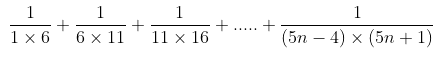Solution:

[1/(1 × 6)] + [1/(6 × 11)] + [1/(11 × 16)] + …. + [1/(5n – 4)(5n + 1)]

Tr = 1/ (5r – 4)(5r + 1)

= (1/5) [1/(5r – 4) – 1/(5r + 1)]

T1 = (1/5)[1 – (1/6)]

T2 = (1/5) [(1/6) – (1/11)]

….

Sn = T1 + T2 + T3 + …. + Tn

Sn = (1/6) – (1/30) + (1/30) – (1/55) + (1/55) +….+ (1/5) [ 1/(5n – 4) – 1/(5n + 1)]

= (1/5) – (1/5) [1/(5n + 1)]

= (1/5) [(5n + 1 – 1)/ (5n + 1)]

= (1/5) [5n/ (5n + 1)]

= n/(5n + 1)

Question 14 

Find the standard deviation for the following data:

 Class 10 – 20 20 – 30 30 – 40 40 – 50 50 – 60 60 – 70 70 – 80 80 – 90 Frequency 8 10 15 25 20 18 9 5

Solution:

 Class Frequency (fi) Class mark (xi) fixi fi(xi)2 10 – 20 8 15 120 1800 20 – 30 10 25 250 6250 30 – 40 15 35 525 18375 40 – 50 25 45 1125 50625 50 – 60 20 55 1100 60500 60 – 70 18 65 1170 76050 70 – 80 9 75 675 50625 80 – 90 5 85 425 36125 Total ∑fi = 110 ∑fixi = 5390 ∑fi(xi)2 = 300350

Variance = 1/(N – 1) [∑fixi2 – (1/N) (∑fixi)2]

= [1/(110 – 1)] [300350 – (5390)2/ 110]

= (1/109) [300350 – 264110]

= 36240/ 109

= 332.477

Standard deviation = √(332.477) = 18.234

SECTION B (20 Marks)

Question 15 [3×2]

(a) At what point of the parabola x2 = 9y is the abscissa three times that of ordinate?

Solution:

Given equation of parabola is:

x2 = 9y

Given that abscissa is three times that of ordinate.

Thus, (3y1, y1) be the point of the given parabola.

Substituting this point in the given equation,

(3y1)2 = 9(y1)

9y12 = 9y1

y12 – y1 = 0

y1(y1 – 1) = 0

y1 = 0 or y1 – 1 = 0

y1 = 0 or y1 = 1

3y1 = 3(1) = 3

Hence, the point is (3, 1).

(b) Construct a truth table for (p v q) ⋀ (~p v ~q).

Solution:

 p q ~p ~q p v q ~p v ~q (p v q) ⋀ (~p v ~q) T T T F T T T T F F T T T T F T T F T T T F F T T F T F

(c) Write Converse and inverse of the given conditional statement:

I go to the beach whenever it is a sunny day.

Solution:

Given conditional statement is:

I go to a beach whenever it is a sunny day.

Converse: If I go to a beach, then it is a sunny day.

Inverse: I do not go to a beach, if it is not a sunny day.

Question 16 

(a) The length of the major axis of an ellipse is 20 units and its foci are (±5√3, 0) Find the equation of the ellipse.

Solution:

We know that,

The general form of equation of an ellipse is x2/a2 + y2/b2 = 1

Where, vertices = (±a, 0) and foci = (±c, 0)

Given,

Foci = (±5√3, 0)

i.e. c = 5√3

Length of the major axis of an ellipse = 20 units

Thus, the length of the semi-major axis = 20/2 = 10 units

⇒ a = 10

Now,

c2 = a2 – b2

(5√3)2 = (10)2 – b2

b2 = 100 – 75 = 25

Therefore, the equation of an ellipse is:

x2/100 + y2/25 = 1

OR

(b) Find the eccentricity, coordinates of the foci, equations of directrices, and length of the latus-rectum of the hyperbola 4x2 – 3y2 = 36.

Solution:

Given equation of hyperbola is:

4x2 – 3y2 = 36

(4x2/36) – (3y2/36) =1

(x2/9) – (y2/12) = 1

This is of the form (x2/a2) – (y2/b2) = 1,

a2 = 9, b2 = 12

Now,

b2 = a2(e2 – 1)

12 = 9(e2 – 1)

e2 – 1 = 12/9

e2 = (4/3) + 1

e2 = (4 + 3)/3

e = √(7/3)

ae = ±3 × (√(7/3)

= ±(√3 × √3) × (√7/ √3)

= ±√21

Therefore, the coordinates of foci are (±ae, 0) = (±√21, 0)

Equation of directrices:

x = ±a/e

= ±3 × (√3/ √7)

√7x = ±3√3

The length of latus-rectum = 2b2/a

= (2 × 12)/3

= 8

Question 17 

(a) Find the ratio in which the line joining (2, 4, 5) and (3, 5, 4) is divided by the yz-plane.

Solution:

We know that the x-coordinate is 0 in the yz-plane.

Let P(0, y, z) divide the line segment joining the points A(2, 4, 5) and B(3, 5, 4) in the ratio k : 1.

Here,

(2, 4, 5) = (x1, y1, z1)

(3, 5, 4) = (x2, y2, z2)

m : n = k : 1

Using the section formula,

P(x, y, z) = [(kx2 + x1)/ (k + 1), (ky2 + y1)/ (k + 1), (kz2 + z1)/ (k + 1)]

(0, y, z) = [(3k + 2)/ (k + 1), (5k + 4)/ (k + 1), (4k + 5)/ (k + 1)]

Now equating the corresponding elements,

(3k + 2)/ (k + 1) = 0

3k + 2 = 0

3k = -2

k = -2/3

Therefore, P divides the given points externally in the ratio 2 : 3.

OR

(b) If the origin is the centroid of a triangle with vertices (2a, 2, 6), (-4, 3b,-10) and (8, 14, 2c), then find the values of a, b, and c.​

Solution:

Let the given vertices of a triangle be:

(2a, 2, 6) = (x1, y1, z1)

(-4, 3b, -10) = (x2, y2, z2)

(8, 14, 2c) = (x3, y3, z3)

Centroid = [(x1 + x2 + x3)/3, (y1 + y2 + y3)/3, (z1 + z2 + z3)/3]

= [(2a – 4 + 8)/ 3, (2 + 3b + 14)/3, (6 – 10 + 2c)/3]

According to the given,

[(4 + 2a)/3, (16 + 3b)/3, (-4 + 2c)/3] = (0, 0, 0)

By equating the corresponding coordinates,

(4 + 2a)/3 = 0

4 + 2a = 0

2a = -4

a = -4/2 = -2

Now,

(16 + 3b)/3 = 0

16 + 3b = 0

3b = -16

b = -16/3

And

(-4 + 2c)/3 = 0

-4 + 2c = 0

2c = 4

c = 4/2 = 2

Therefore, a = -2, b = -16/3, and c = 2.

Question 18 

Find the equation of the parabola whose focus is (-1, 1) and directrix is 4x + 3y – 24 = 0.

Solution:

Given,

Focus = S(-1, 1)

Equation of directrix (M) is 4x + 3y – 24 = 0

Let P(x, y) be any point on the parabola.

Distance from focus to the point = Distance from the directrix to the point

SP = PM

√[(x + 1)2 + (y – 1)2] = (4x + 3y – 24)/ √(42 + 32)

Squaring on both sides,

x2 + 1 + 2x + y2 + 1 – 2y = (4x + 3y – 24)2/ (16 + 9)

25(x2 + y2 + 2x – 2y + 2) = 16x2 + 9y2 + 576 + 24xy – 144y – 192x

25x2 + 25y2 + 50x – 50y + 50 – 16x2 – 9y2 – 24xy + 144y + 192x – 576 = 0

9x2 + 16y2 – 24xy + 242x + 94y – 526 = 0

This is the required equation of parabola.

SECTION C (20 Marks)

Question 19

(a) The mean weight of 100 students in a class is 46 kg. The mean weight of girls is 50 and of the boys is 40 kg. Find the number of girls and boys in the class. 

Solution:

Given,

Mean weight of 100 students = 46 kg

Mean weight of girls = 50 kg

Mean weight of boys = 40 kg

Let x and y be the number of girls and boys in the class respectively.

Thus, x + y = 100

x = 100 – y….(i)

According to the given,

46 = (50x + 40y)/ (x + y)

50x + 40y = 46 × 100

50(100 – y) + 40y = 4600 [From (i)]

5000 – 50y + 40y = 4600

5000 – 4600 = 50y – 40y

10y = 400

y = 400/10

y = 40

Substituting y = 40 in (i),

x = 100 – 40 = 60

Hence, the number of girls are 60 and the number of boys are 40.

(b) Calculate P65 for the following distribution. 

 CI 0 – 10 10 – 20 20 – 30 30 – 40 40 – 50 50 – 60 60 – 70 Frequency 3 10 17 7 6 4 3

Solution:

 Marks (C.I) Frequency Cumulative frequency 0 – 10 3 3 10 – 20 10 13 20 – 30 17 30 30 – 40 7 37 40 – 50 6 43 50 – 60 4 47 60 – 70 3 50

N = 60

P at 65 = p = (65/100) × 50 = 32.5

Cumulative frequency greater than and nearest to 32.5 is 37 which lies in the interval 30 – 40.

Percentile class = 30 – 40

Lower limit of the percentile class = l = 30

Frequency of the percentile class = f = 7

Cumulative frequency of the class preceding the percentile class = cf = 30

Class height = h = 10

P65 = l + [(p – cf)/ f] × h

= 30 + [(32.5 – 30)/ 7] × 10

= 30 + (25/7)

= 30 + 3.57

= 33.57

OR

Find mode from the following distribution.

 Class 0 – 10 10 – 20 20 – 30 30 – 40 40 – 50 50 – 60 Frequency 11 29 18 4 5 3

Solution:

 C.I Frequency 0 – 10 11 10 – 20 29 20 – 30 18 30 – 40 4 40 – 50 5 50 – 60 3

From the given data,

Maximum frequency = 29

Modal class is 10 – 20

Frequency of the modal class = f1 = 29

Frequency of the class preceding the modal class = f0 = 11

Frequency of the class succeeding the modal class = f2 = 18

Lower limit of the modal class = l = 10

Class height = h = 10

Mode = l + [(f1 – f0)/ (2f1 – f0 – f2)] × h

= 10 + [(29 – 11)/ (2 × 29 – 11 – 18)] × 10

= 10 + [18/ (58 – 29)] × 10

= 10 + (180/ 29)

= 10 + 6.207

= 16.207

Question 20

(a) In a sample of ‘n’ observations given that ∑d2 = 22 and rank correlation r = 31/ 42 then find the value of n. 

Solution:

Given,

∑d2 = 22

Rank correlation = r = 31/42

We know that,

Rank correlation = 1 – [(6 × ∑d2)/ (n(n2 – 1)

31/42 = 1 – [(6 × 22)/ (n(n2 – 1)]

⇒ 132/ n(n2 – 1) = 1 – (31/42)

⇒ 132/ n(n2 – 1) = (42 – 31)/ 42

⇒ n(n2 – 1) = (42/11) × 132

⇒ n(n2 – 1) = 504

⇒ n(n2 – 1) = 8 × 63

⇒ n(n2 – 1) = 8 × (64 – 1)

⇒ n(n2 – 1) = 8 × [(8)2 – 1]

⇒ n = 8

(b) Find the correlation coefficient r(x, y) if:

n = 7, ∑x = 19, ∑y = 565, ∑x2 = 75, ∑y2 = 46775, ∑xy = 1380 

Solution:

Given,

n = 7

∑x = 19

∑y = 565

∑x2 = 75

∑y2 = 46775

∑xy = 1380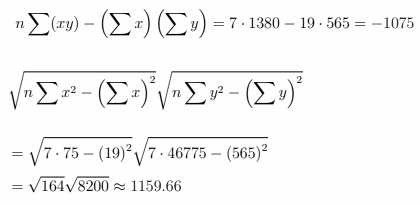Therefore, correlation coefficient = -1075/ 1159.66 = -0.93

OR

Find Spearman’s rank correlation coefficient for the below data.

 X 50 175 270 375 425 580 710 790 890 980 Y 180 120 200 100 100 120 80 60 100 85

Solution:

 X Rank (R1) Y Rank (R2) d = R1 – R2 d2 50 10 180 2 8 64 175 9 120 3.5 5.5 30.25 270 8 200 1 7 49 375 7 100 6 1 1 425 6 100 6 0 0 580 5 120 3.5 1.5 2.25 710 4 80 9 -5 25 790 3 60 10 -7 49 890 2 100 6 -4 16 980 1 85 8 -7 49

n = 10

∑d2 = 285.5

The spearman’s rank correlation coefficient = 1 – [(6 × ∑d2)/ n(n2 – 1)]

= 1 – [(6 × 285.5)/ 10(100 – 1)]

= 1 – [1713/ 10 × 99]

= 1 – (1713/990)

= (990 – 1713)/ 990

= -723/990

= -0.73

Question 21 

Calculate the Index Number for the following data using the Weighted average of price relatives Method for the year 1995 with respect to 1990 as the base.

 Commodity Price in 1995 (in Rs.) Price in 1990 (in Rs.) Weight A 5.20 4.25 30 B 3.75 2.95 40 C 1.95 2.15 15 D 8.10 8.85 15

Solution:

 Commodity Weights (w) Price in Year 1990 (p0) Price in Year 1995 (p1) Price relative I = (p1/p0) × 100 Iw A 30 4.25 5.20 122.35 3670.5 B 40 2.95 3.75 127.12 5084.8 C 15 2.15 1.95 90.7 1360.5 D 15 8.85 8.10 91.53 1372.95 Total ∑w = 100 ∑Iw = 11488.75

Index number of weighted average of price relatives = ∑Iw/ ∑w

= 11488.75/100

= 114.88

Question 22 

Calculate the 5-year moving average for the following data.

 Year 1995 1996 1997 1998 1999 2000 2001 2002 2003 2004 No. of students 332 317 357 392 402 405 410 427 405 438

Solution:

 Year No. of students 5-year moving total 5-year moving average 1995 332 _ 1996 317 _ 1997 357 1800 360 1998 392 1873 374.6 1999 402 1966 393.2 2000 405 2036 407.2 2001 410 2049 409.8 2002 427 2085 417 2003 405 _ 2004 438 _

Graph: